# RS Aggarwal Solutions for Class 9 Chapter 8 -Triangles

A triangle is a shape having three angles and three sides. It is mainly divided on the basis of number of sides and angles it possesses. Different types of triangles based on the number of sides are Scalene triangles, Isosceles triangles, Equilateral triangles and the triangles based on the angles are Obtuse angled triangle, Acute angled triangle and Right angled triangle. Scoring good marks in the exams has become much simpler with the help of RS Aggarwal Solutions for Class 9. The exercise wise solutions with steps based on the evaluation in the Class 9 exams improves confidence in students to solve difficult questions. RS Aggarwal Solutions for Class 9 Chapter 8 Triangles are provided here.

## RS Aggarwal Solutions for Class 9 Chapter 8: Triangles Download PDF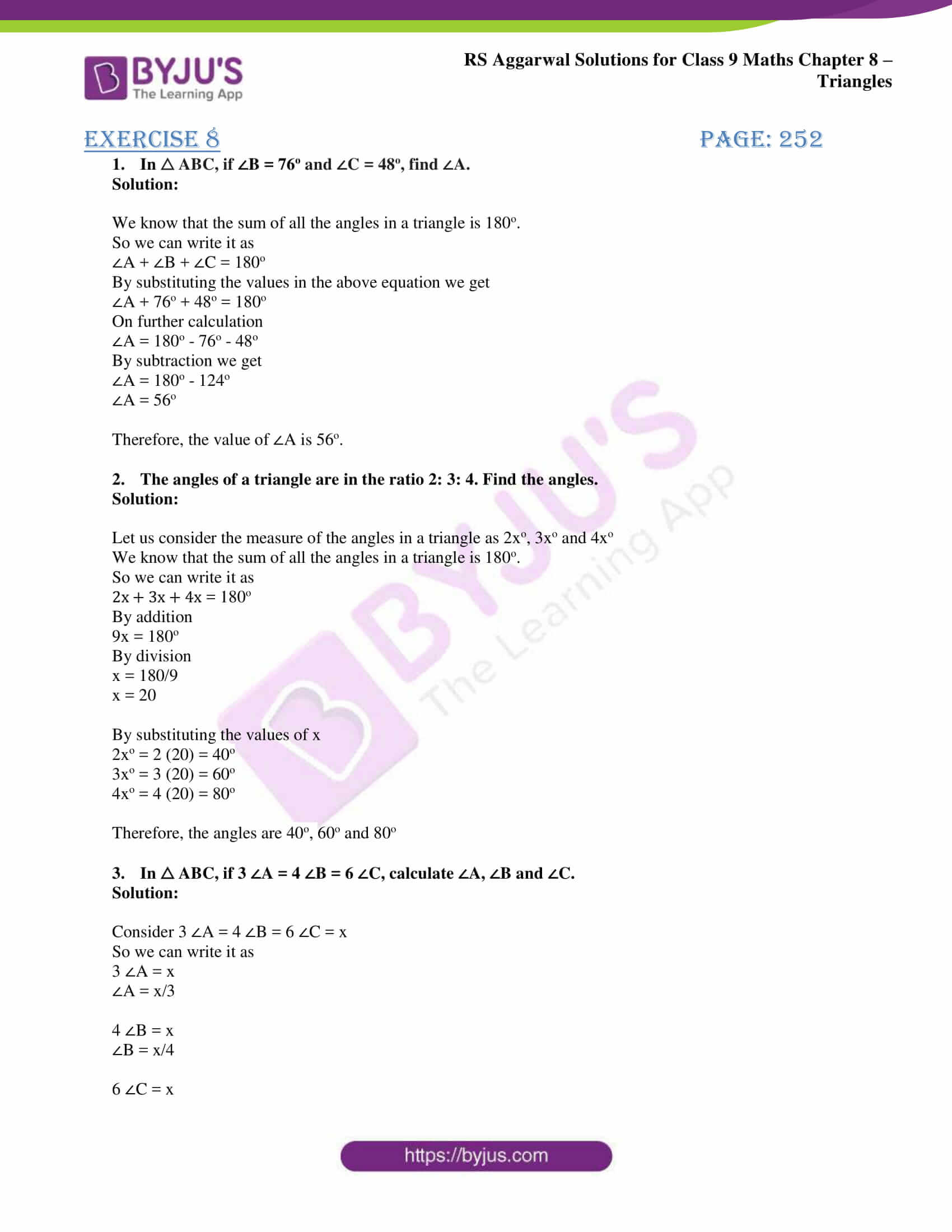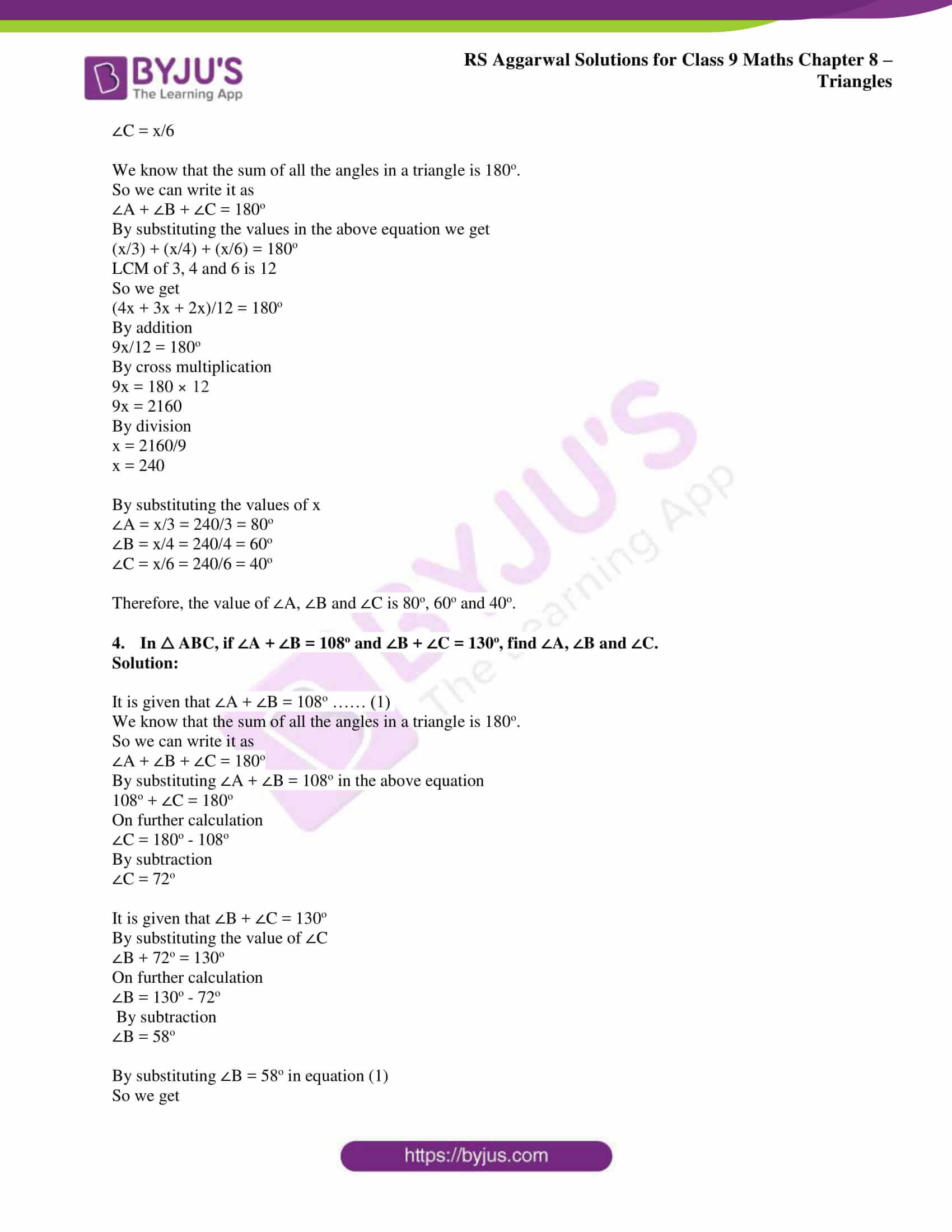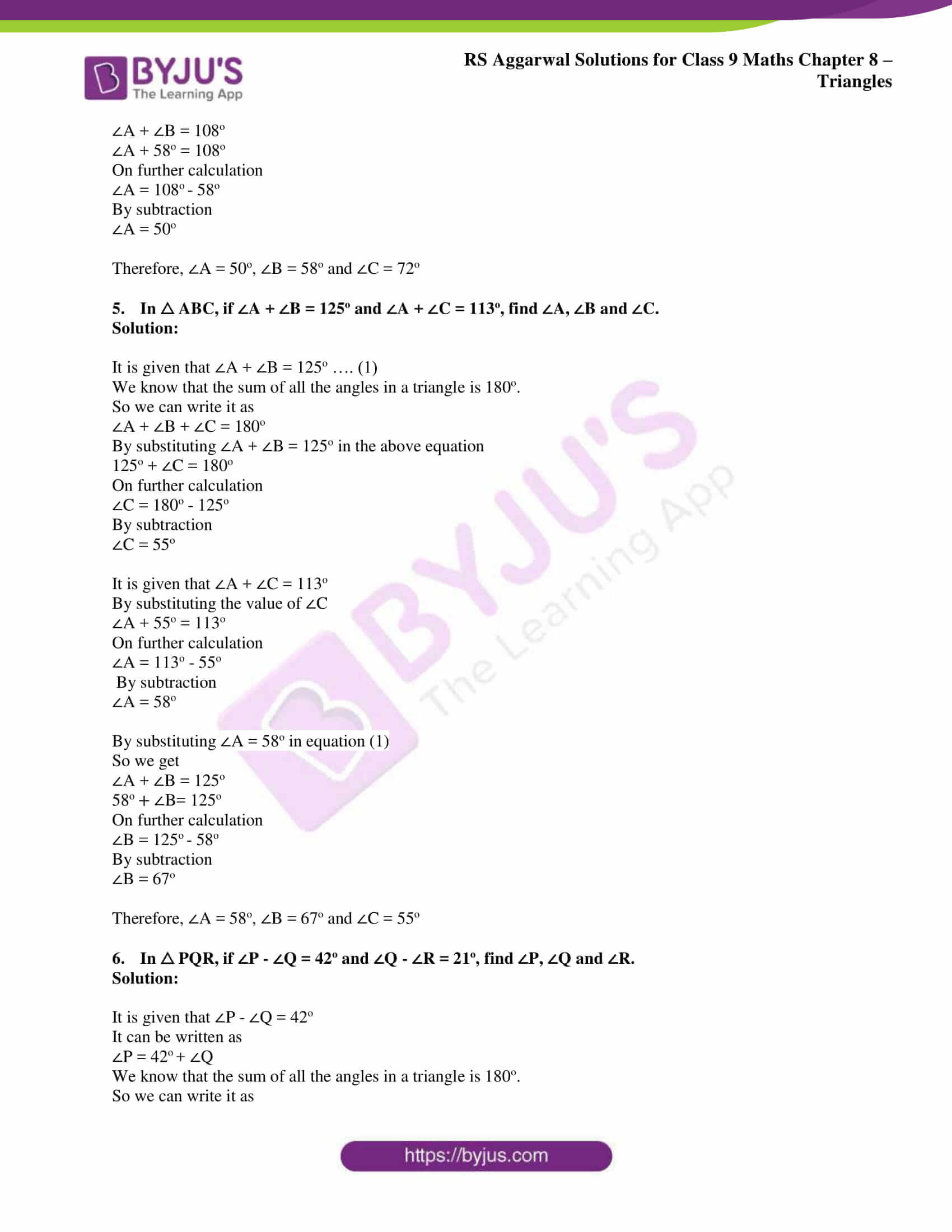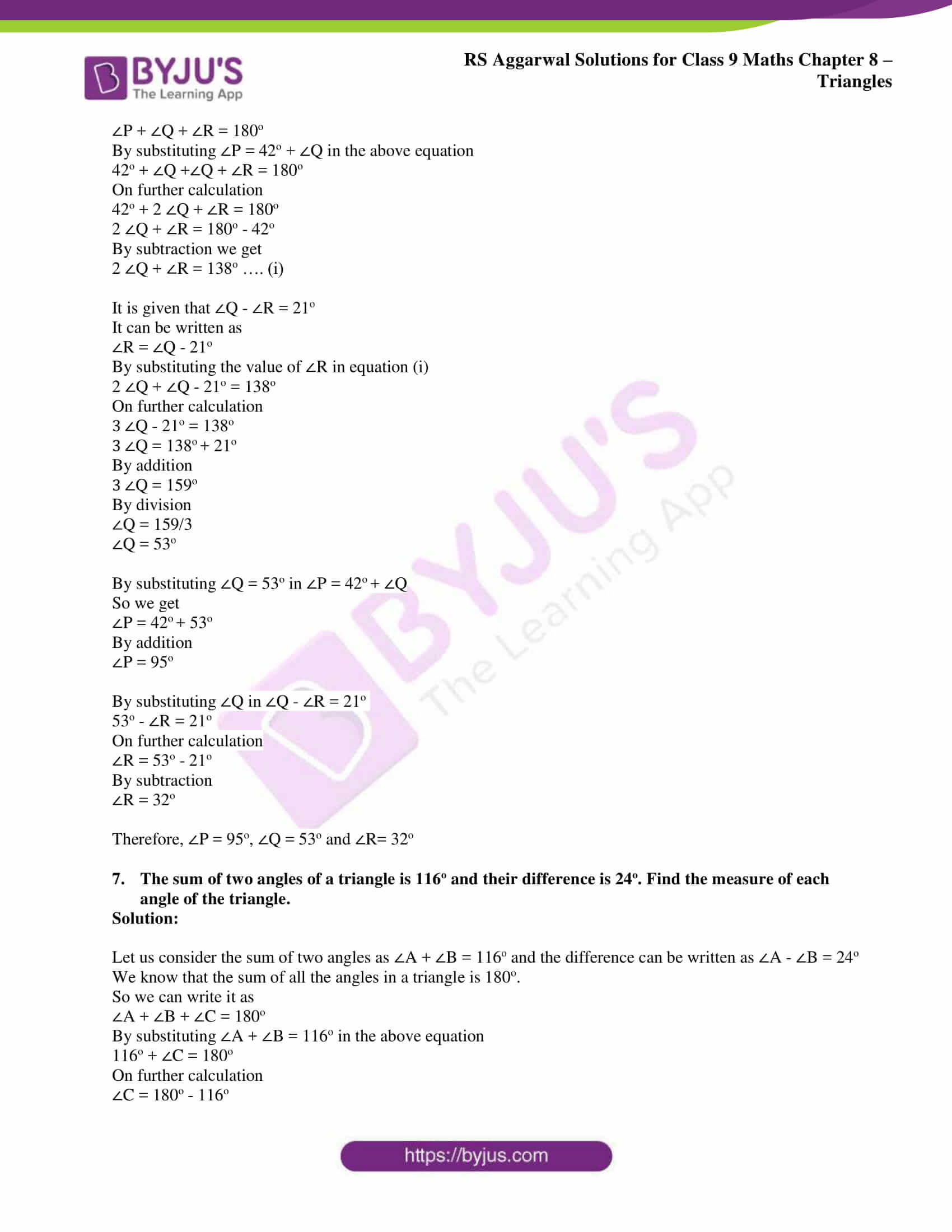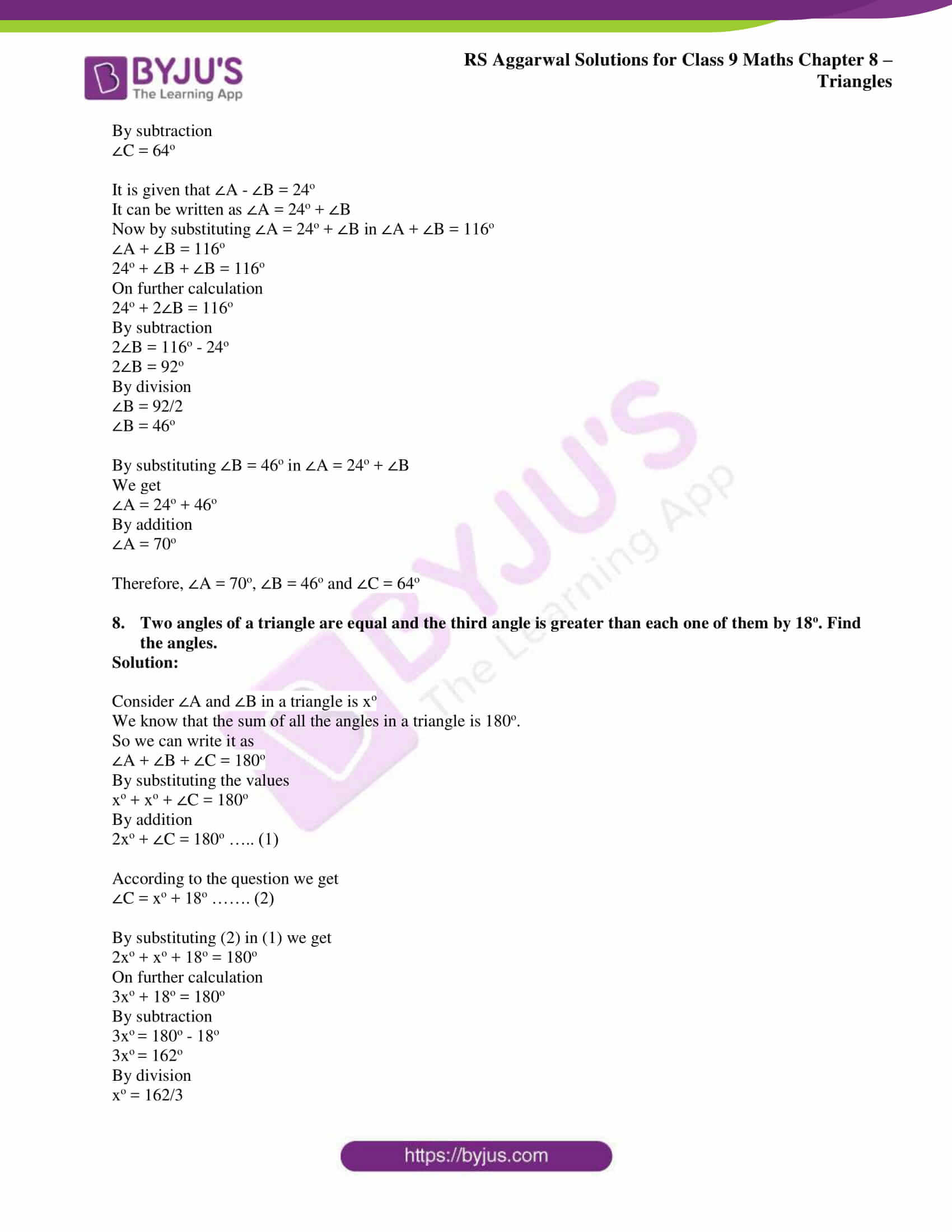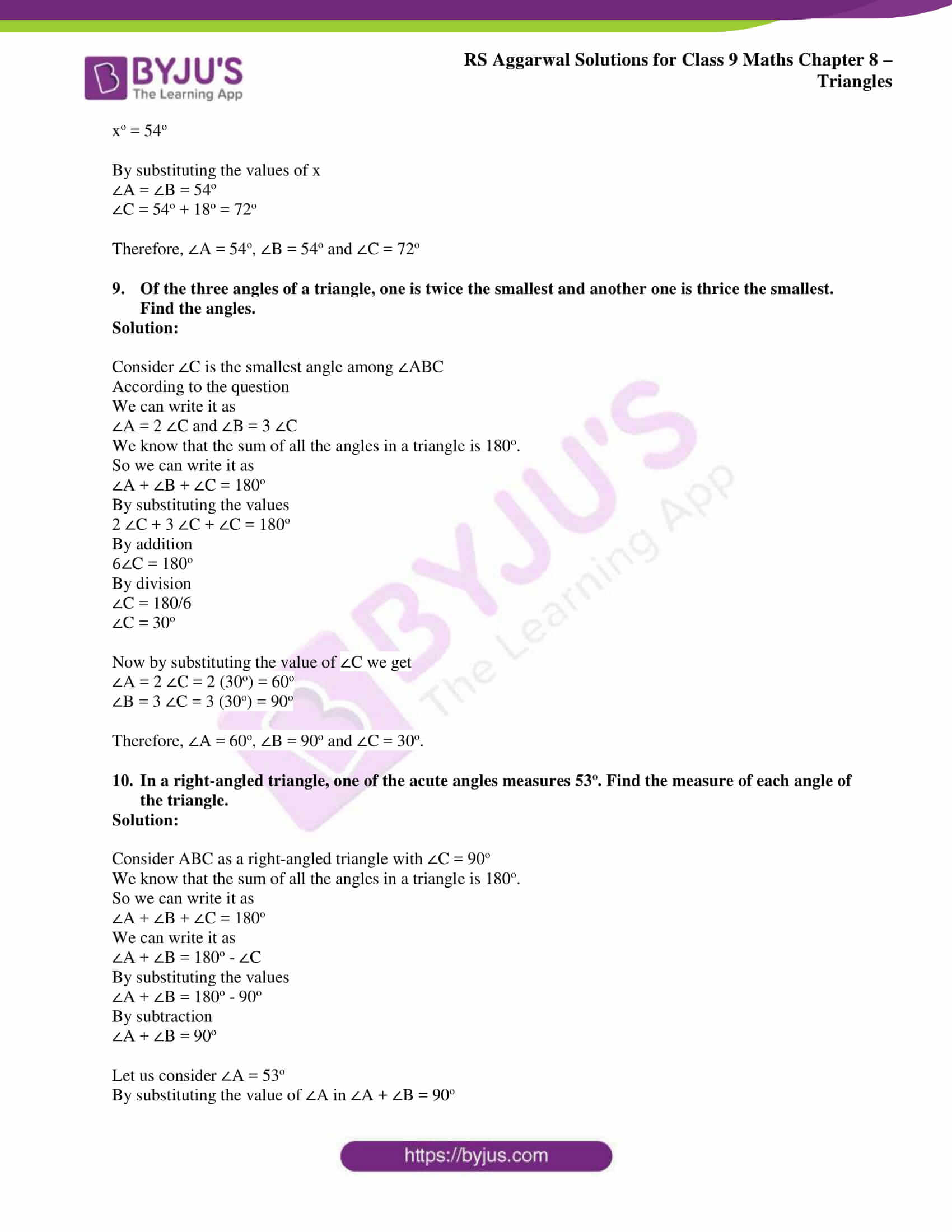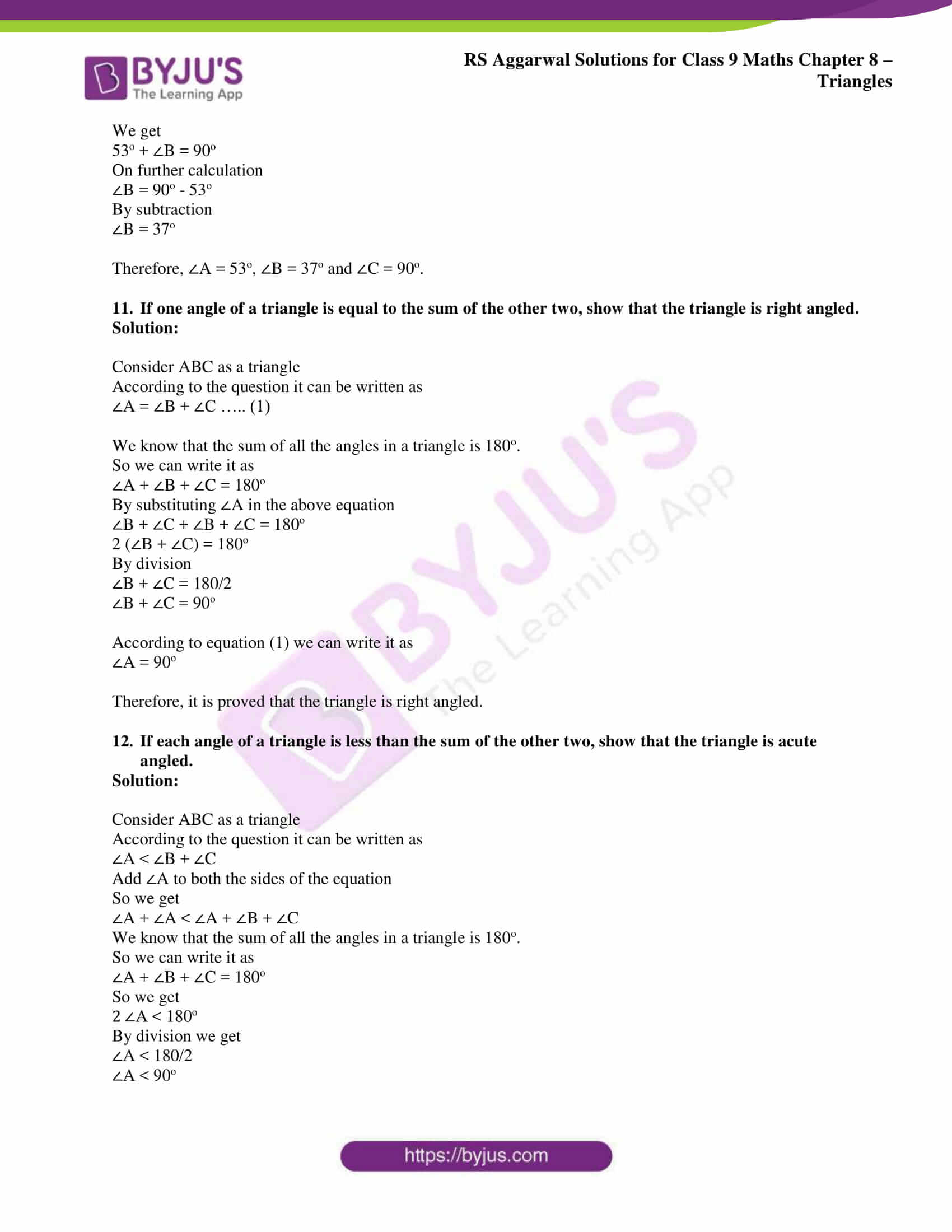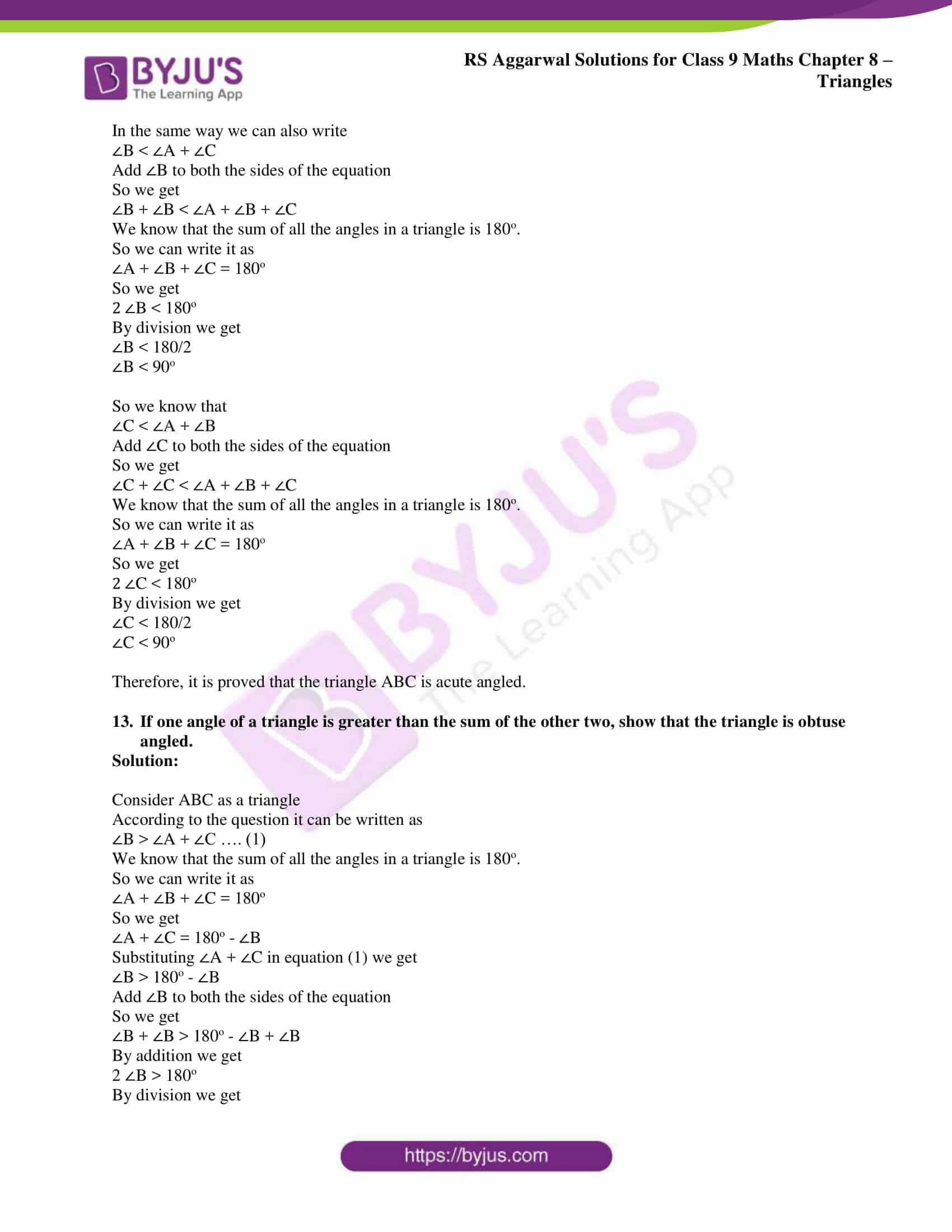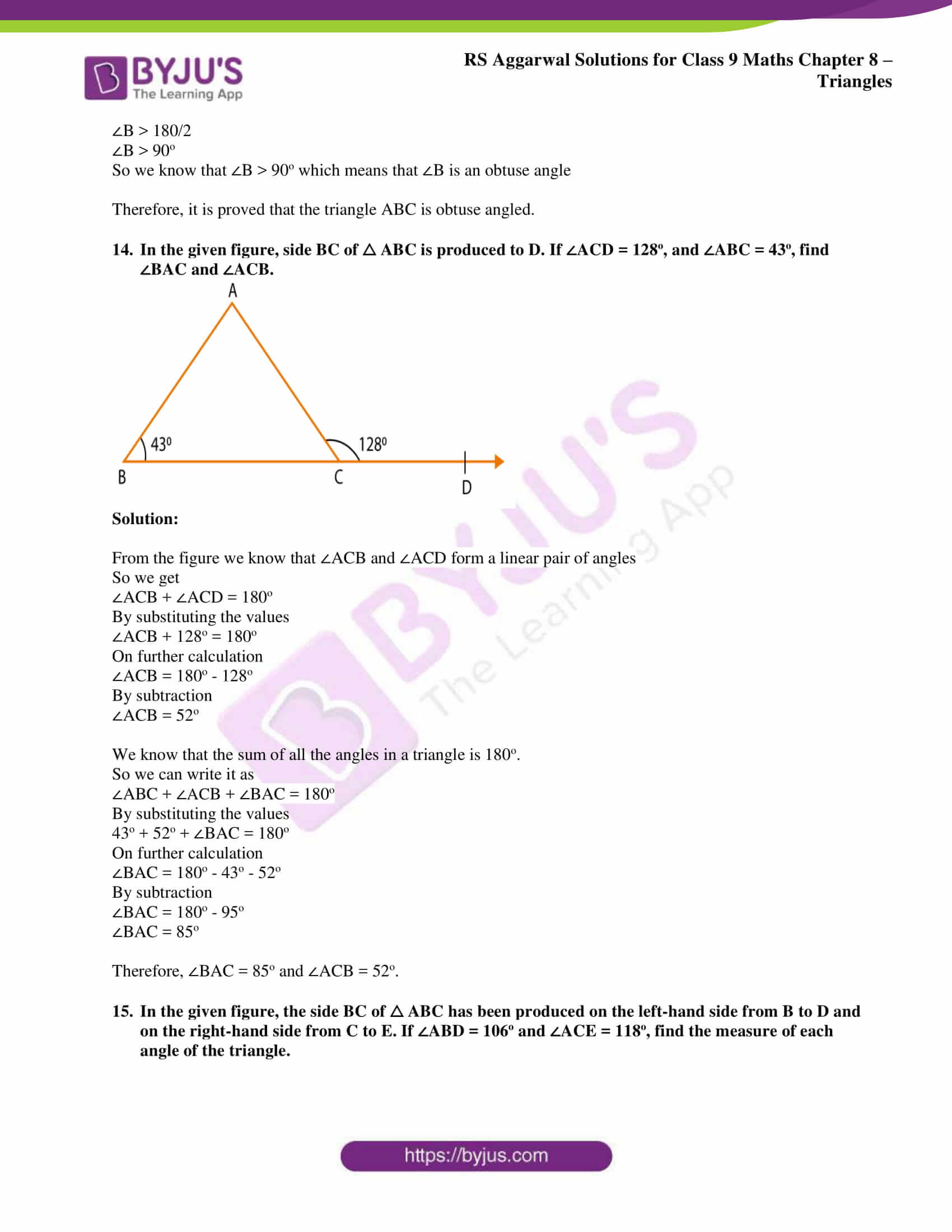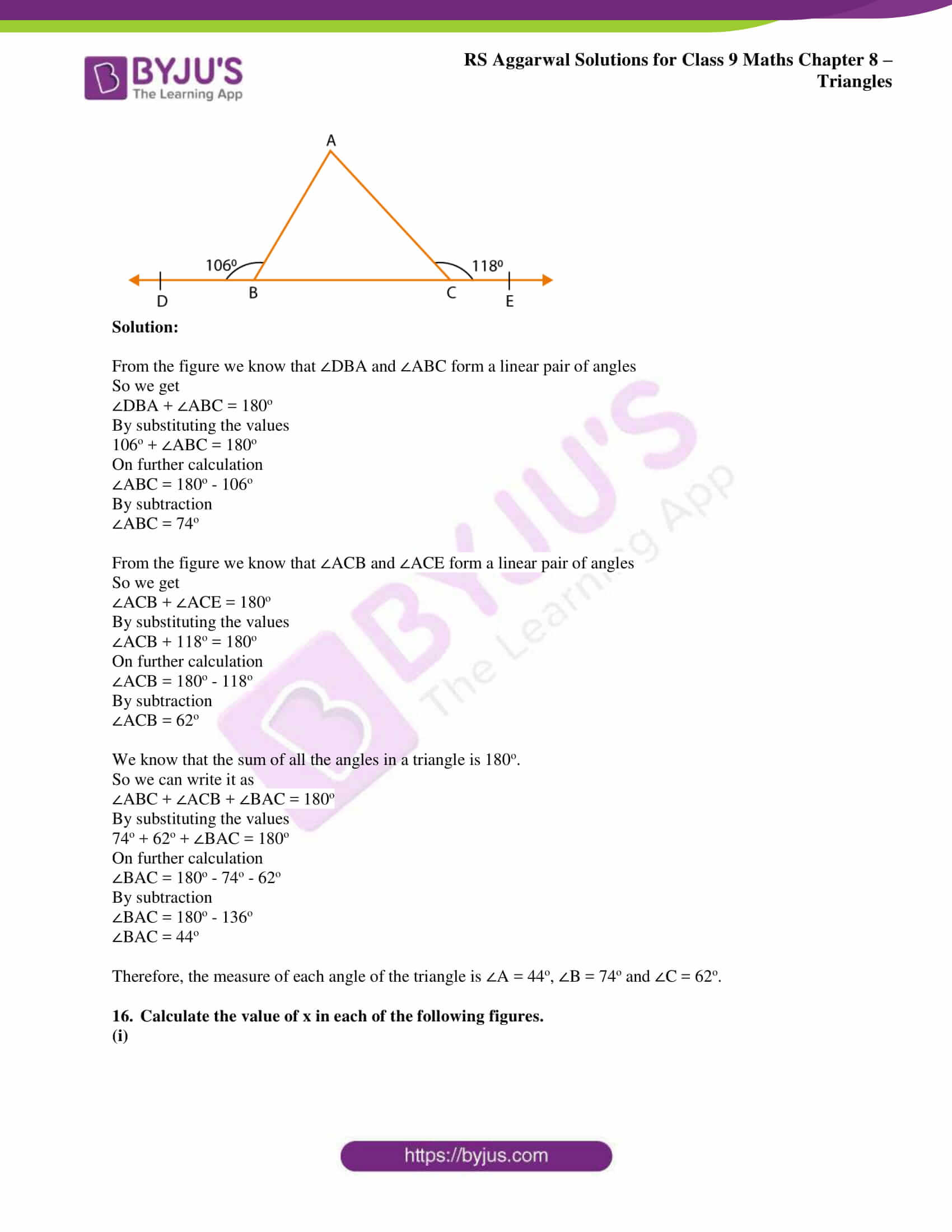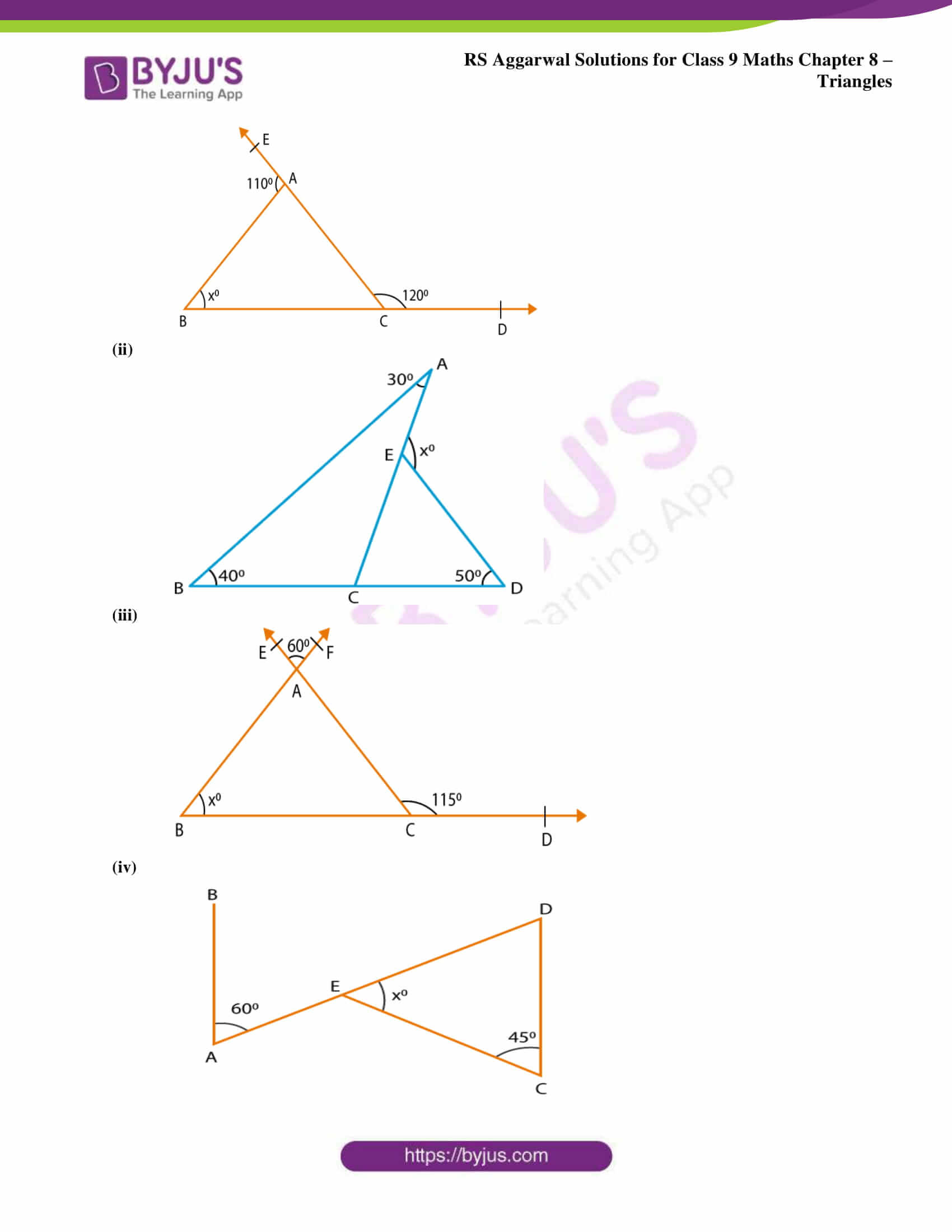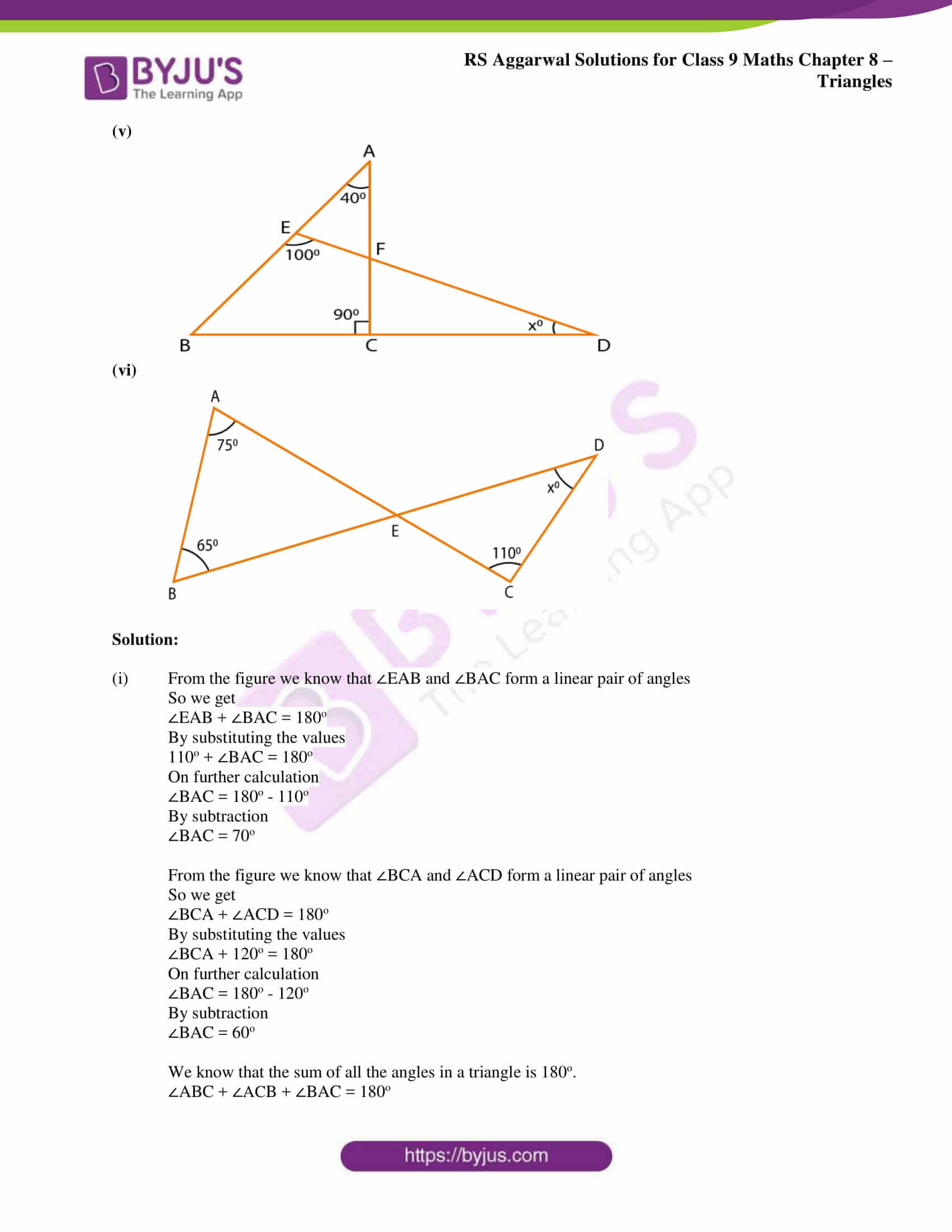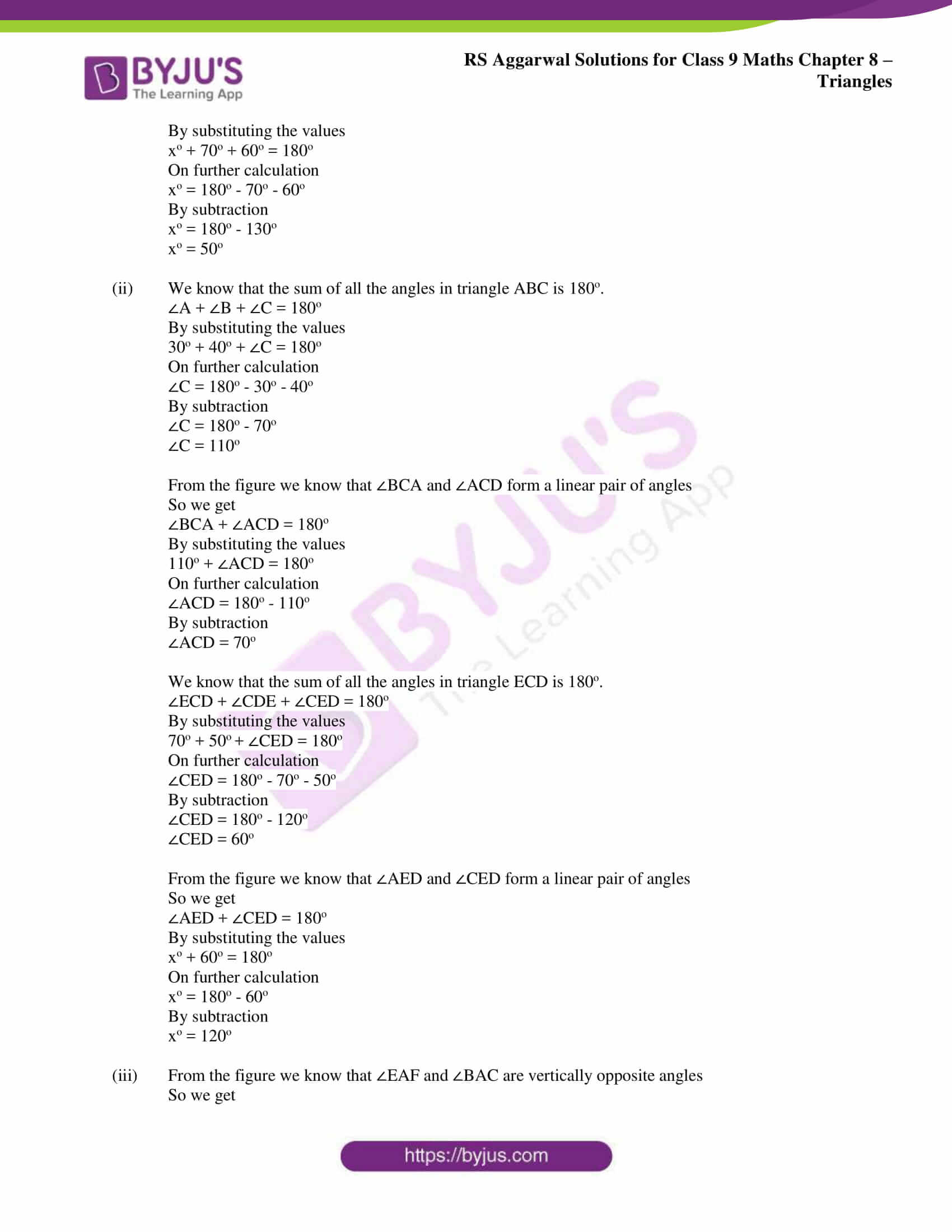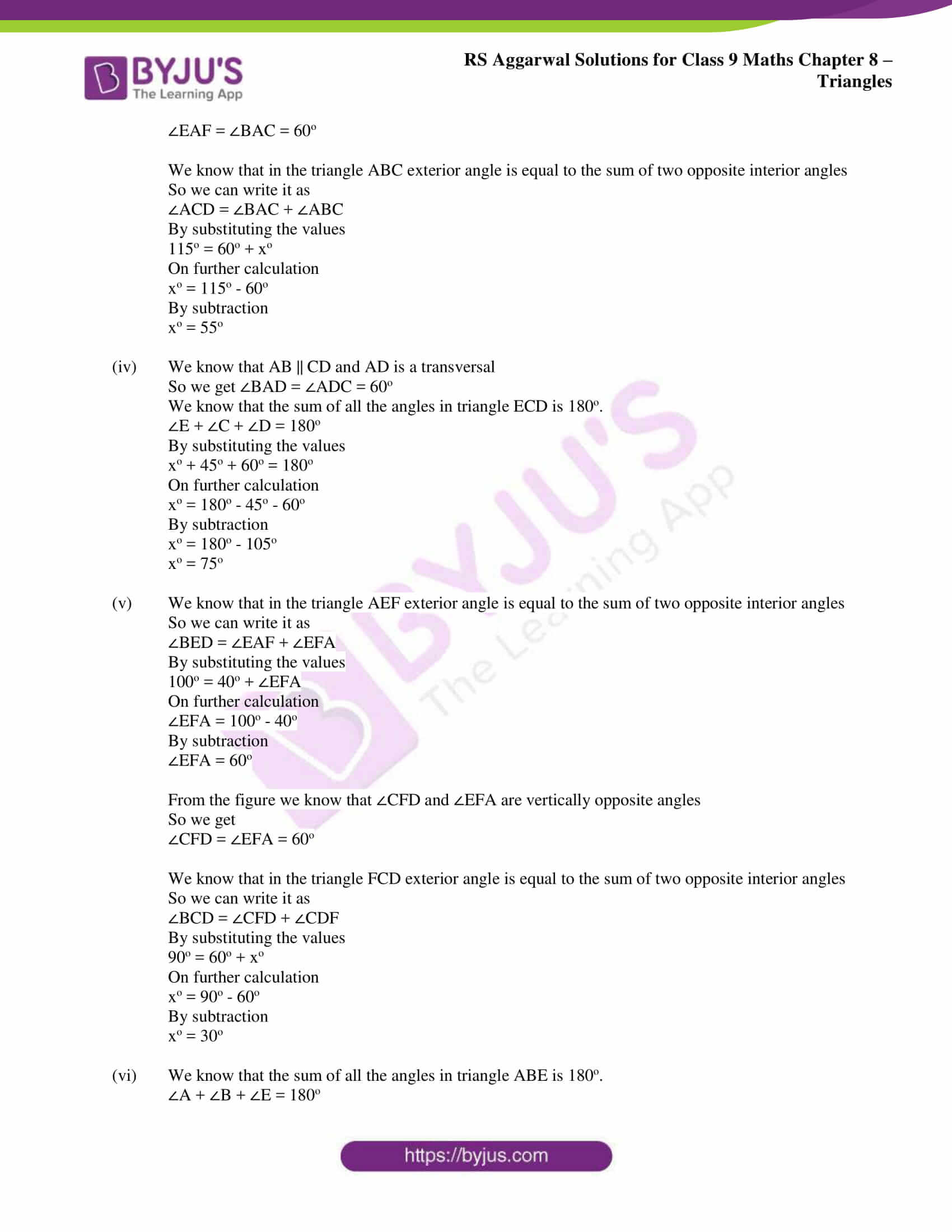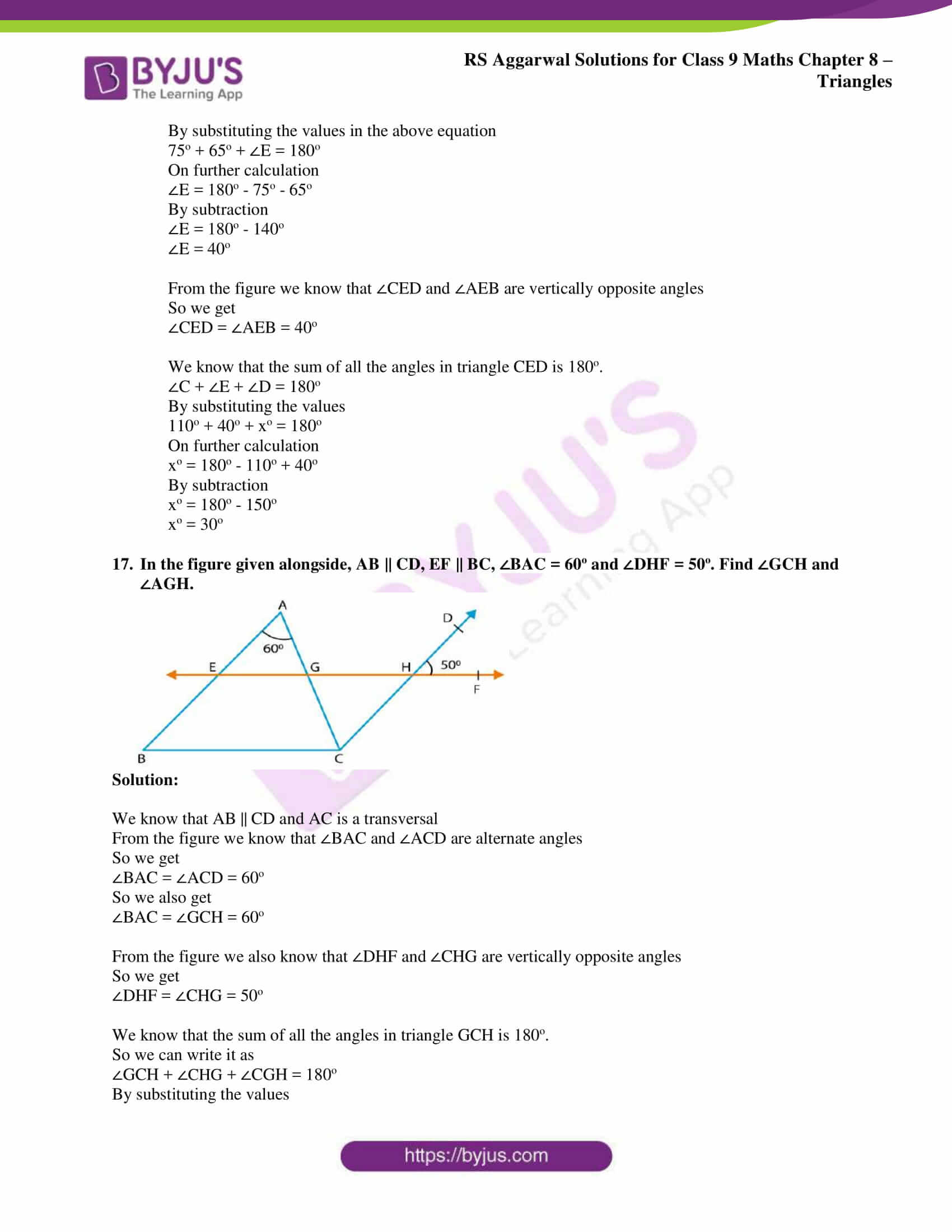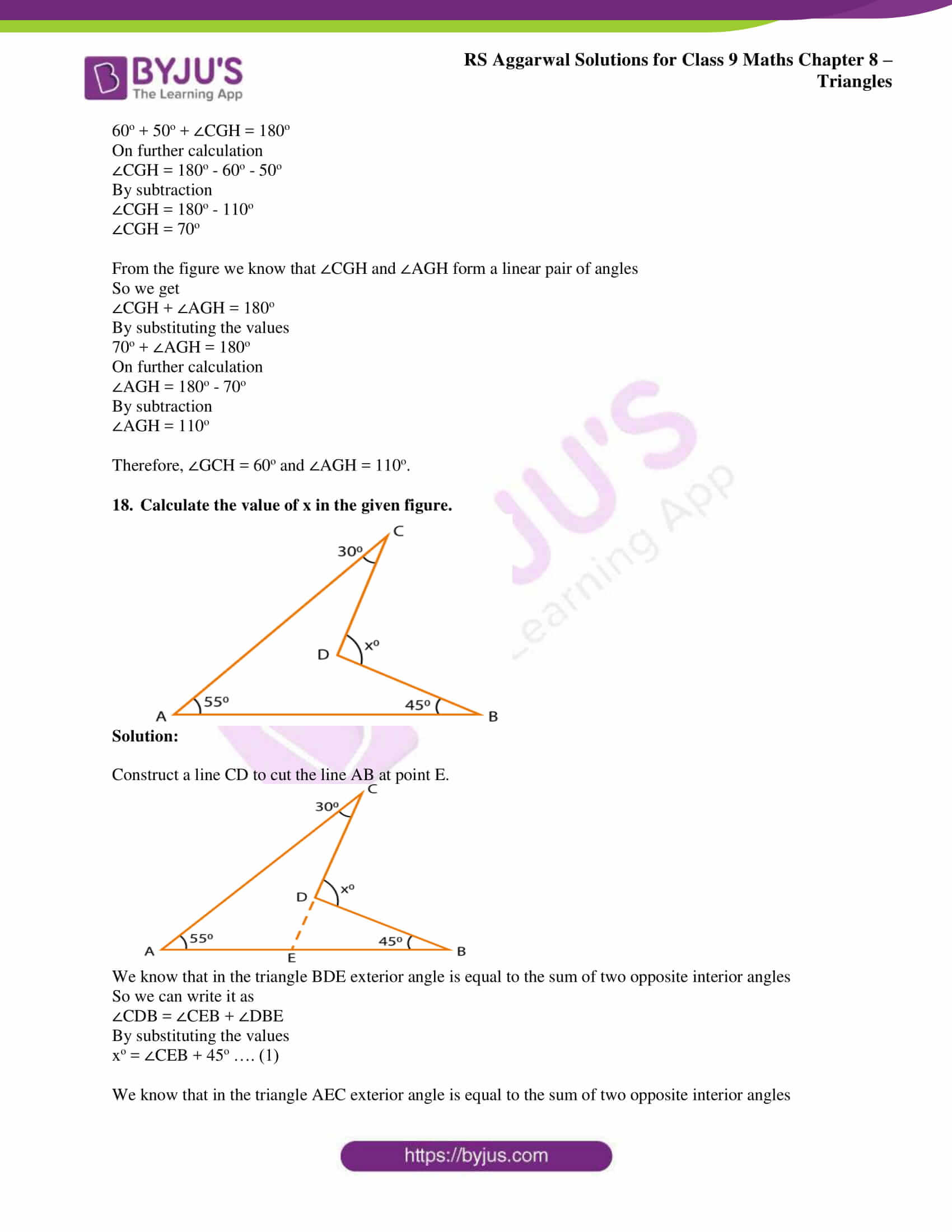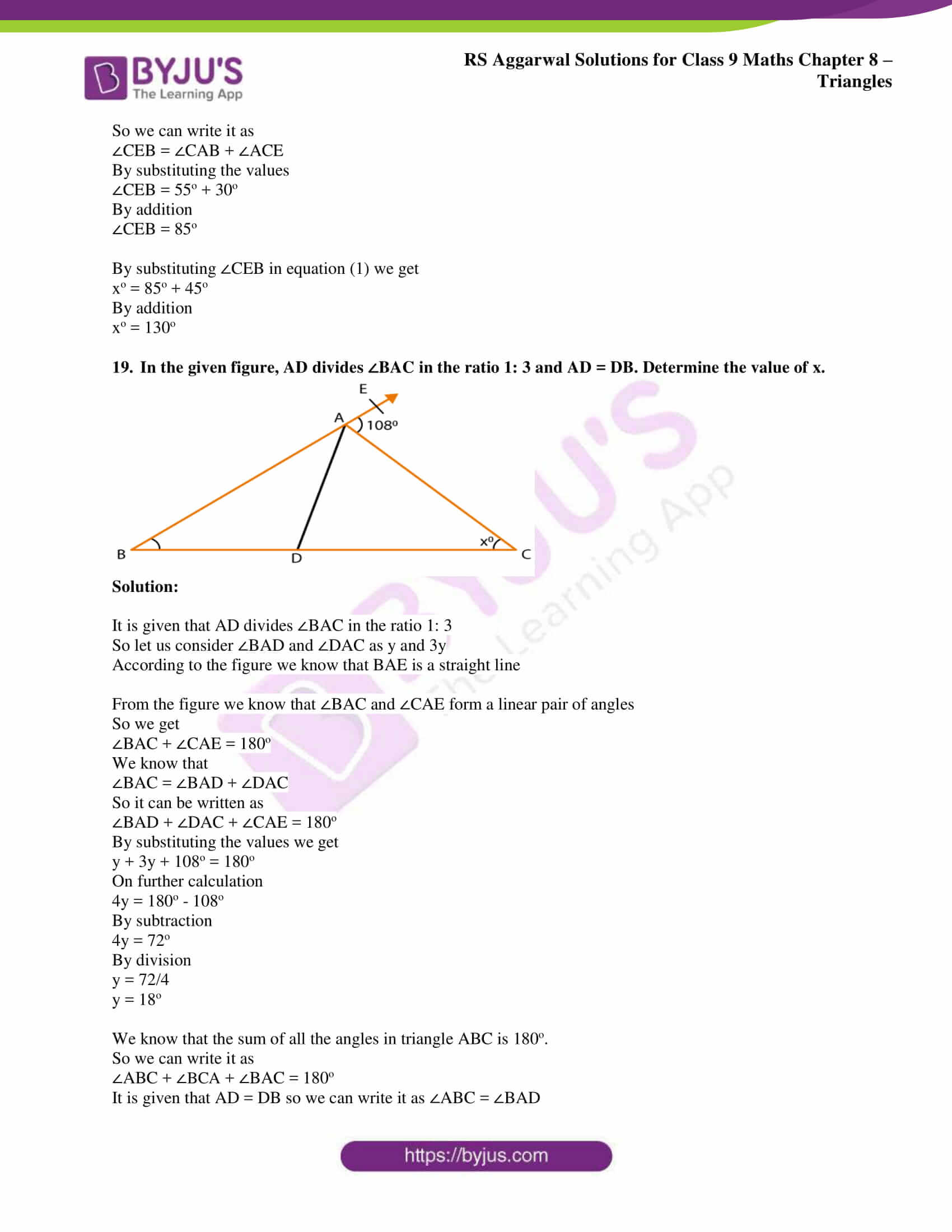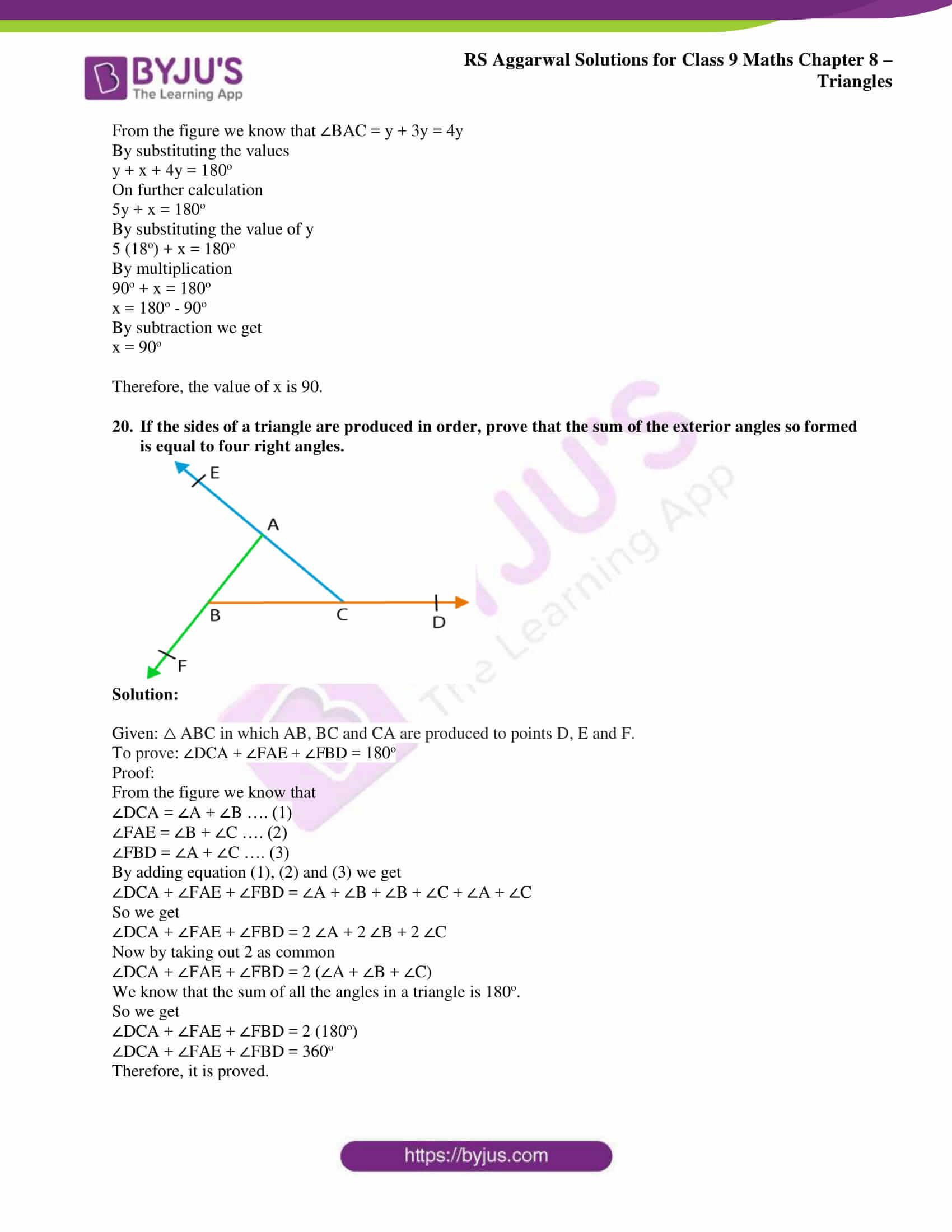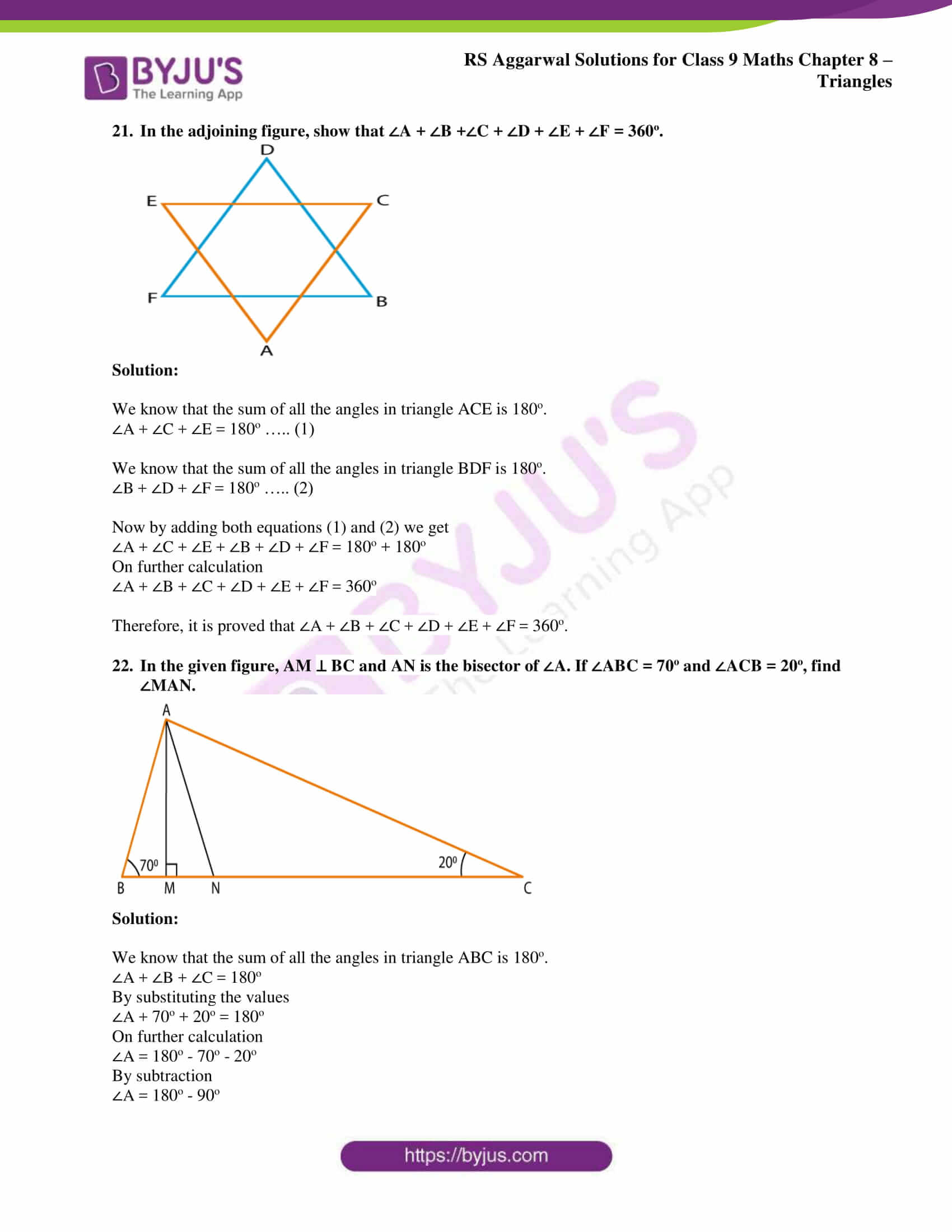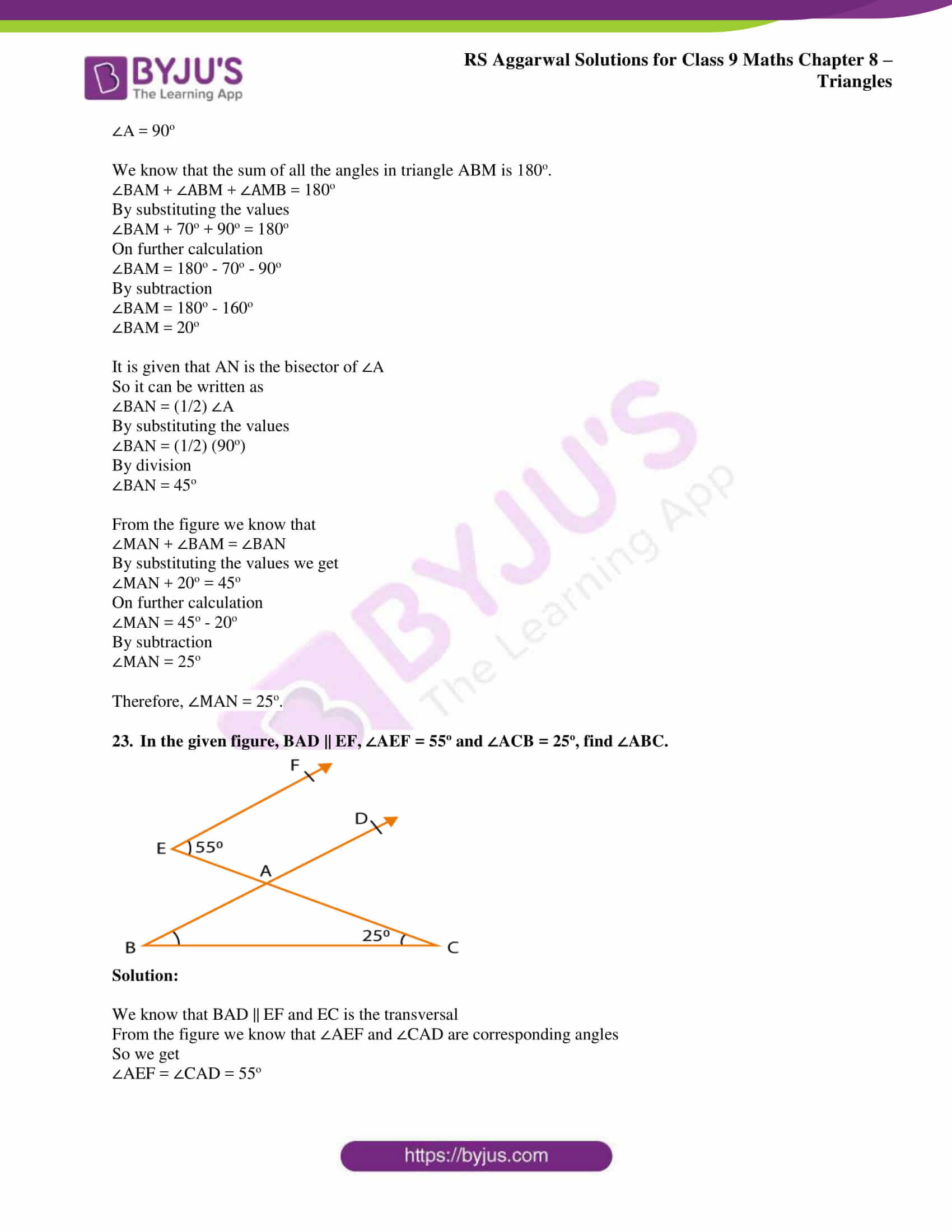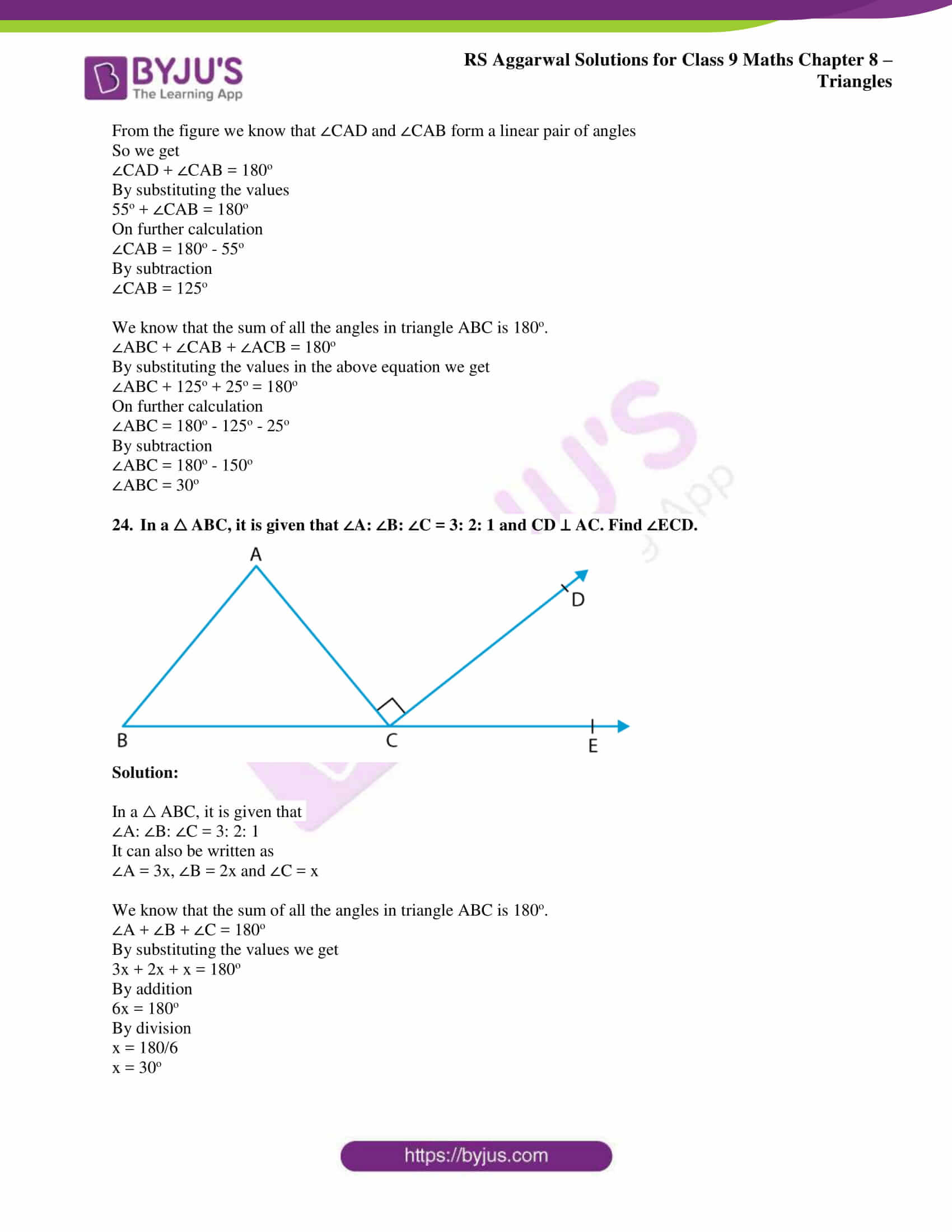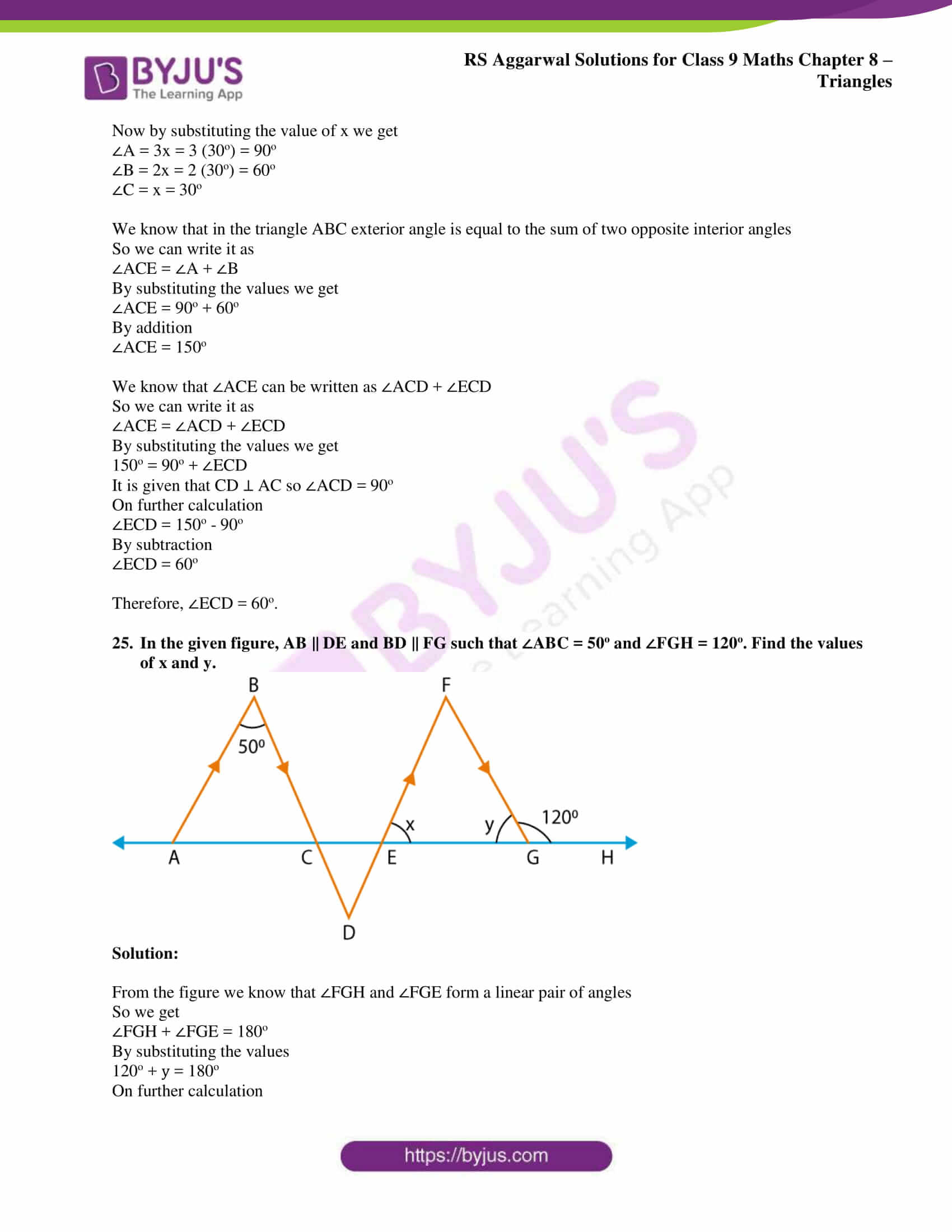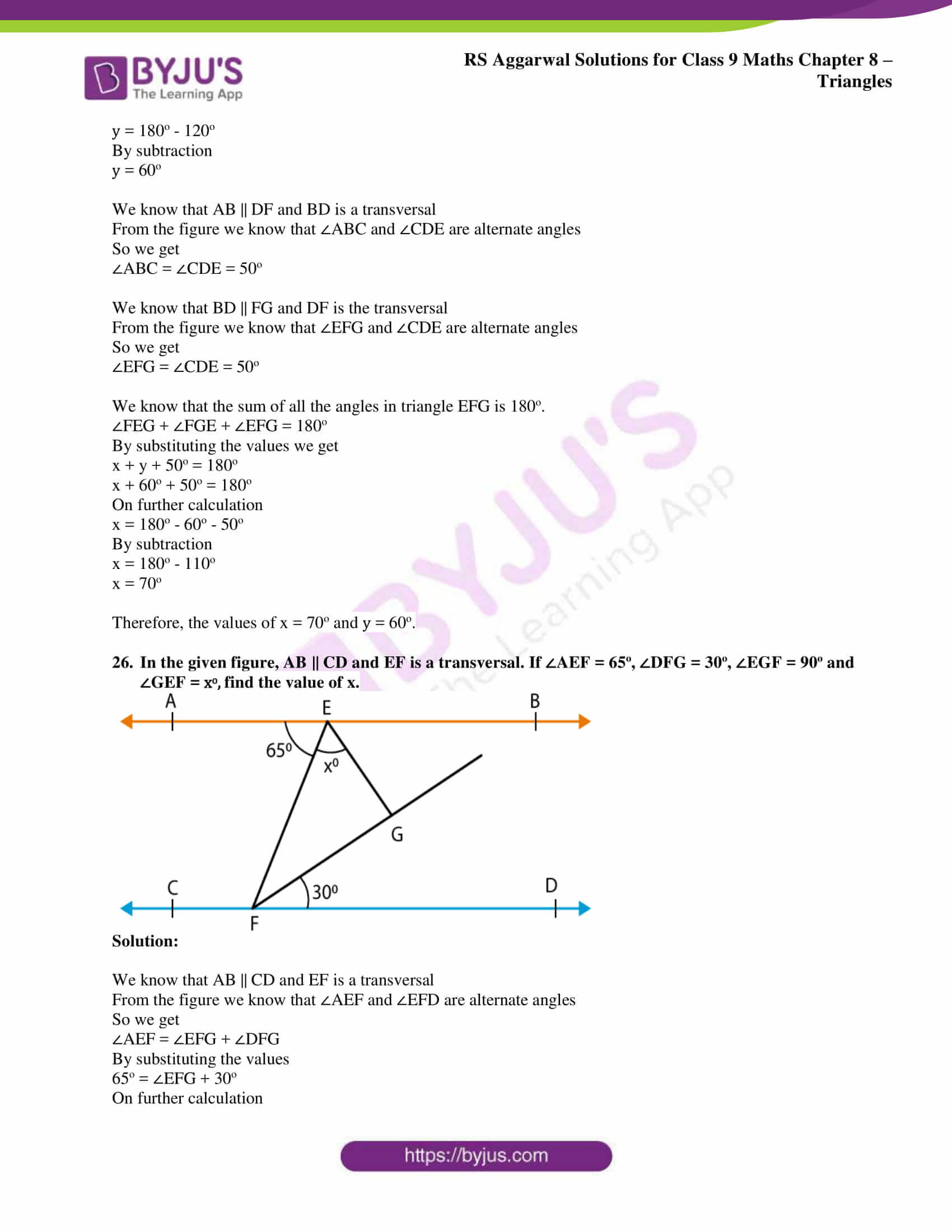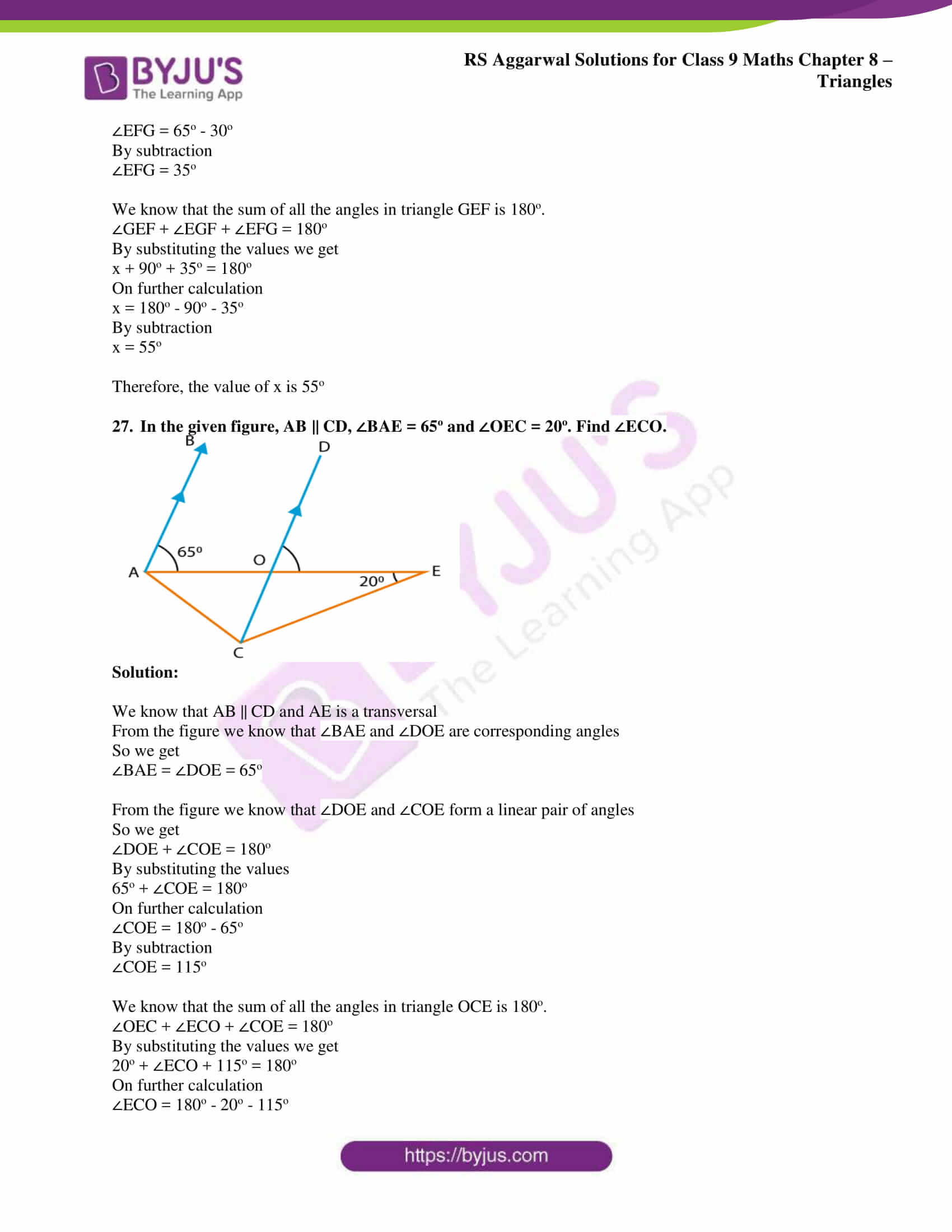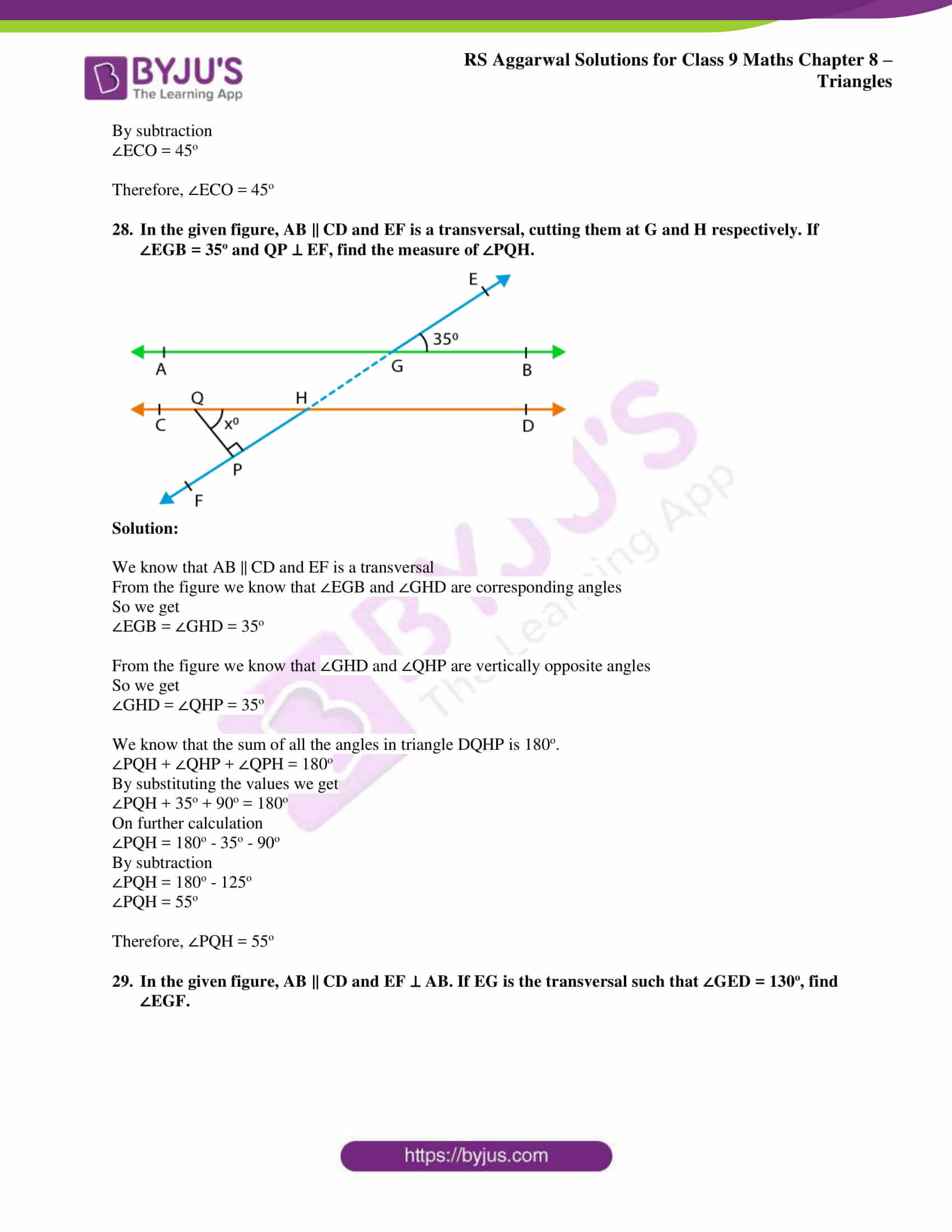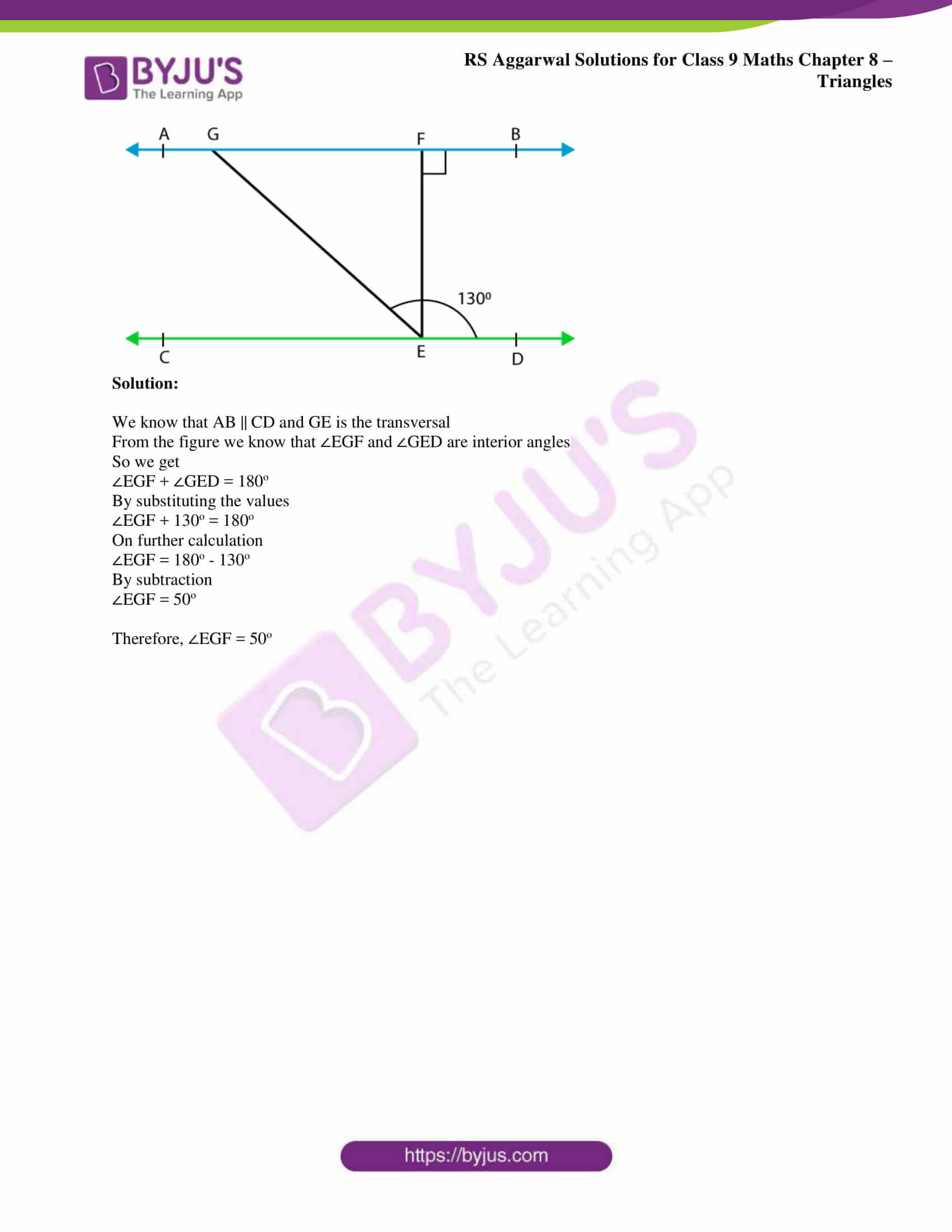## Access RS Aggarwal Solutions for Class 9 Chapter 8: Triangles

Exercise 8 PAGE: 252

1. In △ ABC, if ∠B = 76o and ∠C = 48o, find ∠A.

Solution:

We know that the sum of all the angles in a triangle is 180o.

So we can write it as

∠A + ∠B + ∠C = 180o

By substituting the values in the above equation we get

∠A + 76o + 48o = 180o

On further calculation

∠A = 180o – 76o – 48o

By subtraction we get

∠A = 180o – 124o

∠A = 56o

Therefore, the value of ∠A is 56o.

2. The angles of a triangle are in the ratio 2: 3: 4. Find the angles.

Solution:

Let us consider the measure of the angles in a triangle as 2xo, 3xo and 4xo

We know that the sum of all the angles in a triangle is 180o.

So we can write it as

2x + 3x + 4x = 180o

9x = 180o

By division

x = 180/9

x = 20

By substituting the values of x

2xo = 2 (20) = 40o

3xo = 3 (20) = 60o

4xo = 4 (20) = 80o

Therefore, the angles are 40o, 60o and 80o

3. In △ ABC, if 3 ∠A = 4 ∠B = 6 ∠C, calculate ∠A, ∠B and ∠C.

Solution:

Consider 3 ∠A = 4 ∠B = 6 ∠C = x

So we can write it as

3 ∠A = x

∠A = x/3

4 ∠B = x

∠B = x/4

6 ∠C = x

∠C = x/6

We know that the sum of all the angles in a triangle is 180o.

So we can write it as

∠A + ∠B + ∠C = 180o

By substituting the values in the above equation we get

(x/3) + (x/4) + (x/6) = 180o

LCM of 3, 4 and 6 is 12

So we get

(4x + 3x + 2x)/12 = 180o

9x/12 = 180o

By cross multiplication

9x = 180 × 12

9x = 2160

By division

x = 2160/9

x = 240

By substituting the values of x

∠A = x/3 = 240/3 = 80o

∠B = x/4 = 240/4 = 60o

∠C = x/6 = 240/6 = 40o

Therefore, the value of ∠A, ∠B and ∠C is 80o, 60o and 40o.

4. In △ ABC, if ∠A + ∠B = 108o and ∠B + ∠C = 130o, find ∠A, ∠B and ∠C.

Solution:

It is given that ∠A + ∠B = 108o …… (1)

We know that the sum of all the angles in a triangle is 180o.

So we can write it as

∠A + ∠B + ∠C = 180o

By substituting ∠A + ∠B = 108o in the above equation

108o + ∠C = 180o

On further calculation

∠C = 180o – 108o

By subtraction

∠C = 72o

It is given that ∠B + ∠C = 130o

By substituting the value of ∠C

∠B + 72o = 130o

On further calculation

∠B = 130o – 72o

By subtraction

∠B = 58o

By substituting ∠B = 58o in equation (1)

So we get

∠A + ∠B = 108o

∠A + 58o = 108o

On further calculation

∠A = 108o – 58o

By subtraction

∠A = 50o

Therefore, ∠A = 50o, ∠B = 58o and ∠C = 72o

5. In △ ABC, if ∠A + ∠B = 125o and ∠A + ∠C = 113o, find ∠A, ∠B and ∠C.

Solution:

It is given that ∠A + ∠B = 125o …. (1)

We know that the sum of all the angles in a triangle is 180o.

So we can write it as

∠A + ∠B + ∠C = 180o

By substituting ∠A + ∠B = 125o in the above equation

125o + ∠C = 180o

On further calculation

∠C = 180o – 125o

By subtraction

∠C = 55o

It is given that ∠A + ∠C = 113o

By substituting the value of ∠C

∠A + 55o = 113o

On further calculation

∠A = 113o – 55o

By subtraction

∠A = 58o

By substituting ∠A = 58o in equation (1)

So we get

∠A + ∠B = 125o

58o + ∠B= 125o

On further calculation

∠B = 125o – 58o

By subtraction

∠B = 67o

Therefore, ∠A = 58o, ∠B = 67o and ∠C = 55o

6. In △ PQR, if ∠P – ∠Q = 42o and ∠Q – ∠R = 21o, find ∠P, ∠Q and ∠R.

Solution:

It is given that ∠P – ∠Q = 42o

It can be written as

∠P = 42o + ∠Q

We know that the sum of all the angles in a triangle is 180o.

So we can write it as

∠P + ∠Q + ∠R = 180o

By substituting ∠P = 42o + ∠Q in the above equation

42o + ∠Q +∠Q + ∠R = 180o

On further calculation

42o + 2 ∠Q + ∠R = 180o

2 ∠Q + ∠R = 180o – 42o

By subtraction we get

2 ∠Q + ∠R = 138o …. (i)

It is given that ∠Q – ∠R = 21o

It can be written as

∠R = ∠Q – 21o

By substituting the value of ∠R in equation (i)

2 ∠Q + ∠Q – 21o = 138o

On further calculation

3 ∠Q – 21o = 138o

3 ∠Q = 138o + 21o

3 ∠Q = 159o

By division

∠Q = 159/3

∠Q = 53o

By substituting ∠Q = 53o in ∠P = 42o + ∠Q

So we get

∠P = 42o + 53o

∠P = 95o

By substituting ∠Q in ∠Q – ∠R = 21o

53o – ∠R = 21o

On further calculation

∠R = 53o – 21o

By subtraction

∠R = 32o

Therefore, ∠P = 95o, ∠Q = 53o and ∠R= 32o

7. The sum of two angles of a triangle is 116o and their difference is 24o. Find the measure of each angle of the triangle.

Solution:

Let us consider the sum of two angles as ∠A + ∠B = 116o and the difference can be written as ∠A – ∠B = 24o

We know that the sum of all the angles in a triangle is 180o.

So we can write it as

∠A + ∠B + ∠C = 180o

By substituting ∠A + ∠B = 116o in the above equation

116o + ∠C = 180o

On further calculation

∠C = 180o – 116o

By subtraction

∠C = 64o

It is given that ∠A – ∠B = 24o

It can be written as ∠A = 24o + ∠B

Now by substituting ∠A = 24o + ∠B in ∠A + ∠B = 116o

∠A + ∠B = 116o

24o + ∠B + ∠B = 116o

On further calculation

24o + 2∠B = 116o

By subtraction

2∠B = 116o – 24o

2∠B = 92o

By division

∠B = 92/2

∠B = 46o

By substituting ∠B = 46o in ∠A = 24o + ∠B

We get

∠A = 24o + 46o

∠A = 70o

Therefore, ∠A = 70o, ∠B = 46o and ∠C = 64o

8. Two angles of a triangle are equal and the third angle is greater than each one of them by 18o. Find the angles.

Solution:

Consider ∠A and ∠B in a triangle is xo

We know that the sum of all the angles in a triangle is 180o.

So we can write it as

∠A + ∠B + ∠C = 180o

By substituting the values

xo + xo + ∠C = 180o

2xo + ∠C = 180o ….. (1)

According to the question we get

∠C = xo + 18o ……. (2)

By substituting (2) in (1) we get

2xo + xo + 18o = 180o

On further calculation

3xo + 18o = 180o

By subtraction

3xo = 180o – 18o

3xo = 162o

By division

xo = 162/3

xo = 54o

By substituting the values of x

∠A = ∠B = 54o

∠C = 54o + 18o = 72o

Therefore, ∠A = 54o, ∠B = 54o and ∠C = 72o

9. Of the three angles of a triangle, one is twice the smallest and another one is thrice the smallest. Find the angles.

Solution:

Consider ∠C is the smallest angle among ∠ABC

According to the question

We can write it as

∠A = 2 ∠C and ∠B = 3 ∠C

We know that the sum of all the angles in a triangle is 180o.

So we can write it as

∠A + ∠B + ∠C = 180o

By substituting the values

2 ∠C + 3 ∠C + ∠C = 180o

6∠C = 180o

By division

∠C = 180/6

∠C = 30o

Now by substituting the value of ∠C we get

∠A = 2 ∠C = 2 (30o) = 60o

∠B = 3 ∠C = 3 (30o) = 90o

Therefore, ∠A = 60o, ∠B = 90o and ∠C = 30o.

10. In a right-angled triangle, one of the acute angles measures 53o. Find the measure of each angle of the triangle.

Solution:

Consider ABC as a right-angled triangle with ∠C = 90o

We know that the sum of all the angles in a triangle is 180o.

So we can write it as

∠A + ∠B + ∠C = 180o

We can write it as

∠A + ∠B = 180o – ∠C

By substituting the values

∠A + ∠B = 180o – 90o

By subtraction

∠A + ∠B = 90o

Let us consider ∠A = 53o

By substituting the value of ∠A in ∠A + ∠B = 90o

We get

53o + ∠B = 90o

On further calculation

∠B = 90o – 53o

By subtraction

∠B = 37o

Therefore, ∠A = 53o, ∠B = 37o and ∠C = 90o.

11. If one angle of a triangle is equal to the sum of the other two, show that the triangle is right angled.

Solution:

Consider ABC as a triangle

According to the question it can be written as

∠A = ∠B + ∠C ….. (1)

We know that the sum of all the angles in a triangle is 180o.

So we can write it as

∠A + ∠B + ∠C = 180o

By substituting ∠A in the above equation

∠B + ∠C + ∠B + ∠C = 180o

2 (∠B + ∠C) = 180o

By division

∠B + ∠C = 180/2

∠B + ∠C = 90o

According to equation (1) we can write it as

∠A = 90o

Therefore, it is proved that the triangle is right angled.

12. If each angle of a triangle is less than the sum of the other two, show that the triangle is acute angled.

Solution:

Consider ABC as a triangle

According to the question it can be written as

∠A < ∠B + ∠C

Add ∠A to both the sides of the equation

So we get

∠A + ∠A < ∠A + ∠B + ∠C

We know that the sum of all the angles in a triangle is 180o.

So we can write it as

∠A + ∠B + ∠C = 180o

So we get

2 ∠A < 180o

By division we get

∠A < 180/2

∠A < 90o

In the same way we can also write

∠B < ∠A + ∠C

Add ∠B to both the sides of the equation

So we get

∠B + ∠B < ∠A + ∠B + ∠C

We know that the sum of all the angles in a triangle is 180o.

So we can write it as

∠A + ∠B + ∠C = 180o

So we get

2 ∠B < 180o

By division we get

∠B < 180/2

∠B < 90o

So we know that

∠C < ∠A + ∠B

Add ∠C to both the sides of the equation

So we get

∠C + ∠C < ∠A + ∠B + ∠C

We know that the sum of all the angles in a triangle is 180o.

So we can write it as

∠A + ∠B + ∠C = 180o

So we get

2 ∠C < 180o

By division we get

∠C < 180/2

∠C < 90o

Therefore, it is proved that the triangle ABC is acute angled.

13. If one angle of a triangle is greater than the sum of the other two, show that the triangle is obtuse angled.

Solution:

Consider ABC as a triangle

According to the question it can be written as

∠B > ∠A + ∠C …. (1)

We know that the sum of all the angles in a triangle is 180o.

So we can write it as

∠A + ∠B + ∠C = 180o

So we get

∠A + ∠C = 180o – ∠B

Substituting ∠A + ∠C in equation (1) we get

∠B > 180o – ∠B

Add ∠B to both the sides of the equation

So we get

∠B + ∠B > 180o – ∠B + ∠B

2 ∠B > 180o

By division we get

∠B > 180/2

∠B > 90o

So we know that ∠B > 90o which means that ∠B is an obtuse angle

Therefore, it is proved that the triangle ABC is obtuse angled.

14. In the given figure, side BC of △ ABC is produced to D. If ∠ACD = 128o, and ∠ABC = 43o, find ∠BAC and ∠ACB.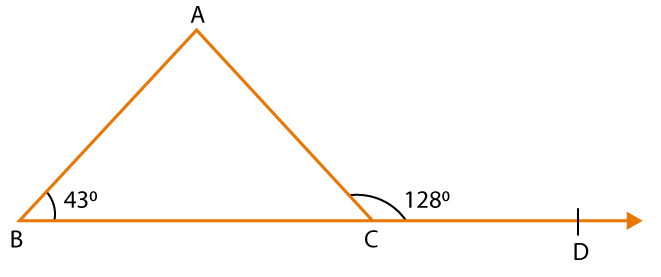Solution:

From the figure we know that ∠ACB and ∠ACD form a linear pair of angles

So we get

∠ACB + ∠ACD = 180o

By substituting the values

∠ACB + 128o = 180o

On further calculation

∠ACB = 180o – 128o

By subtraction

∠ACB = 52o

We know that the sum of all the angles in a triangle is 180o.

So we can write it as

∠ABC + ∠ACB + ∠BAC = 180o

By substituting the values

43o + 52o + ∠BAC = 180o

On further calculation

∠BAC = 180o – 43o – 52o

By subtraction

∠BAC = 180o – 95o

∠BAC = 85o

Therefore, ∠BAC = 85o and ∠ACB = 52o.

15. In the given figure, the side BC of △ ABC has been produced on the left-hand side from B to D and on the right-hand side from C to E. If ∠ABD = 106o and ∠ACE = 118o, find the measure of each angle of the triangle.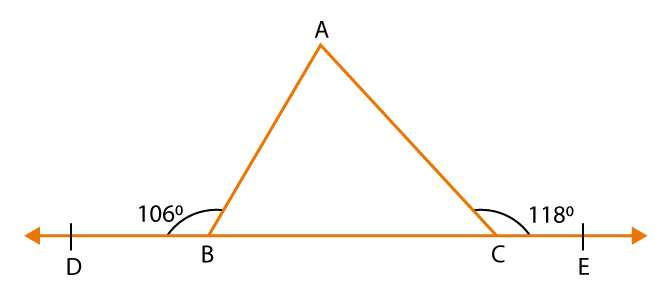Solution:

From the figure we know that ∠DBA and ∠ABC form a linear pair of angles

So we get

∠DBA + ∠ABC = 180o

By substituting the values

106o + ∠ABC = 180o

On further calculation

∠ABC = 180o – 106o

By subtraction

∠ABC = 74o

From the figure we know that ∠ACB and ∠ACE form a linear pair of angles

So we get

∠ACB + ∠ACE = 180o

By substituting the values

∠ACB + 118o = 180o

On further calculation

∠ACB = 180o – 118o

By subtraction

∠ACB = 62o

We know that the sum of all the angles in a triangle is 180o.

So we can write it as

∠ABC + ∠ACB + ∠BAC = 180o

By substituting the values

74o + 62o + ∠BAC = 180o

On further calculation

∠BAC = 180o – 74o – 62o

By subtraction

∠BAC = 180o – 136o

∠BAC = 44o

Therefore, the measure of each angle of the triangle is ∠A = 44o, ∠B = 74o and ∠C = 62o.

16. Calculate the value of x in each of the following figures.

(i)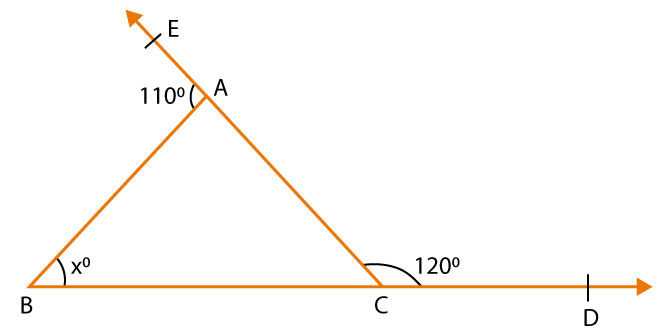(ii)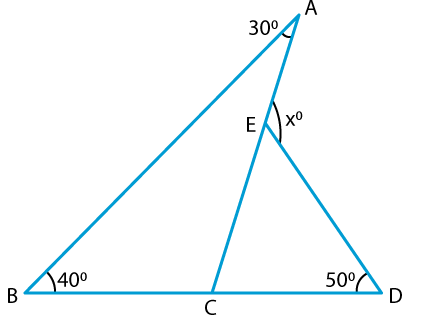(iii)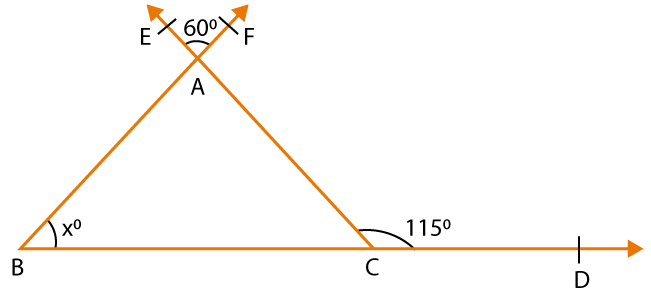(iv)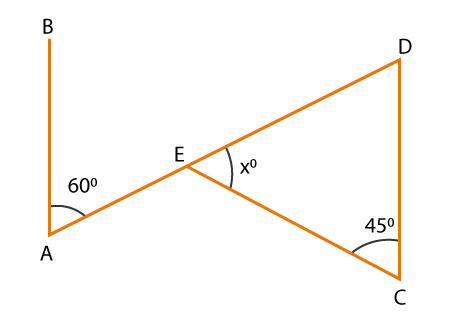(v)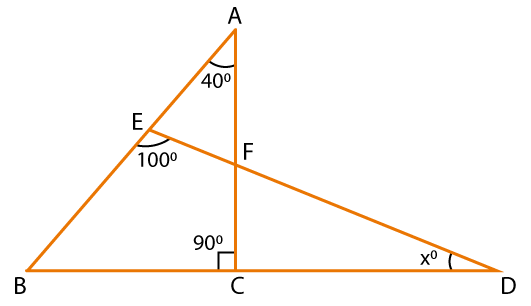(vi)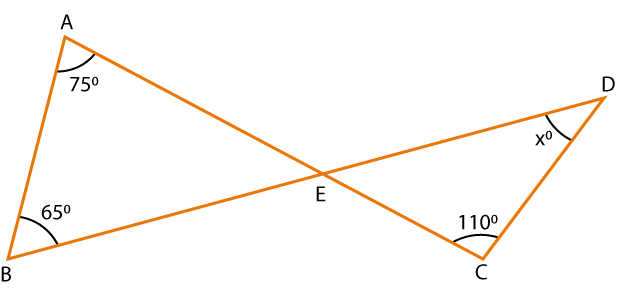Solution:

(i) From the figure we know that ∠EAB and ∠BAC form a linear pair of angles

So we get

∠EAB + ∠BAC = 180o

By substituting the values

110o + ∠BAC = 180o

On further calculation

∠BAC = 180o – 110o

By subtraction

∠BAC = 70o

From the figure we know that ∠BCA and ∠ACD form a linear pair of angles

So we get

∠BCA + ∠ACD = 180o

By substituting the values

∠BCA + 120o = 180o

On further calculation

∠BAC = 180o – 120o

By subtraction

∠BAC = 60o

We know that the sum of all the angles in a triangle is 180o.

∠ABC + ∠ACB + ∠BAC = 180o

By substituting the values

xo + 70o + 60o = 180o

On further calculation

xo = 180o – 70o – 60o

By subtraction

xo = 180o – 130o

xo = 50o

(ii) We know that the sum of all the angles in triangle ABC is 180o.

∠A + ∠B + ∠C = 180o

By substituting the values

30o + 40o + ∠C = 180o

On further calculation

∠C = 180o – 30o – 40o

By subtraction

∠C = 180o – 70o

∠C = 110o

From the figure we know that ∠BCA and ∠ACD form a linear pair of angles

So we get

∠BCA + ∠ACD = 180o

By substituting the values

110o + ∠ACD = 180o

On further calculation

∠ACD = 180o – 110o

By subtraction

∠ACD = 70o

We know that the sum of all the angles in triangle ECD is 180o.

∠ECD + ∠CDE + ∠CED = 180o

By substituting the values

70o + 50o + ∠CED = 180o

On further calculation

∠CED = 180o – 70o – 50o

By subtraction

∠CED = 180o – 120o

∠CED = 60o

From the figure we know that ∠AED and ∠CED form a linear pair of angles

So we get

∠AED + ∠CED = 180o

By substituting the values

xo + 60o = 180o

On further calculation

xo = 180o – 60o

By subtraction

xo = 120o

(iii) From the figure we know that ∠EAF and ∠BAC are vertically opposite angles

So we get

∠EAF = ∠BAC = 60o

We know that in the triangle ABC exterior angle is equal to the sum of two opposite interior angles

So we can write it as

∠ACD = ∠BAC + ∠ABC

By substituting the values

115o = 60o + xo

On further calculation

xo = 115o – 60o

By subtraction

xo = 55o

(iv) We know that AB || CD and AD is a transversal

We know that the sum of all the angles in triangle ECD is 180o.

∠E + ∠C + ∠D = 180o

By substituting the values

xo + 45o + 60o = 180o

On further calculation

xo = 180o – 45o – 60o

By subtraction

xo = 180o – 105o

xo = 75o

(v) We know that in the triangle AEF exterior angle is equal to the sum of two opposite interior angles

So we can write it as

∠BED = ∠EAF + ∠EFA

By substituting the values

100o = 40o + ∠EFA

On further calculation

∠EFA = 100o – 40o

By subtraction

∠EFA = 60o

From the figure we know that ∠CFD and ∠EFA are vertically opposite angles

So we get

∠CFD = ∠EFA = 60o

We know that in the triangle FCD exterior angle is equal to the sum of two opposite interior angles

So we can write it as

∠BCD = ∠CFD + ∠CDF

By substituting the values

90o = 60o + xo

On further calculation

xo = 90o – 60o

By subtraction

xo = 30o

(vi) We know that the sum of all the angles in triangle ABE is 180o.

∠A + ∠B + ∠E = 180o

By substituting the values in the above equation

75o + 65o + ∠E = 180o

On further calculation

∠E = 180o – 75o – 65o

By subtraction

∠E = 180o – 140o

∠E = 40o

From the figure we know that ∠CED and ∠AEB are vertically opposite angles

So we get

∠CED = ∠AEB = 40o

We know that the sum of all the angles in triangle CED is 180o.

∠C + ∠E + ∠D = 180o

By substituting the values

110o + 40o + xo = 180o

On further calculation

xo = 180o – 110o + 40o

By subtraction

xo = 180o – 150o

xo = 30o

17. In the figure given alongside, AB || CD, EF || BC, ∠BAC = 60o and ∠DHF = 50o. Find ∠GCH and ∠AGH.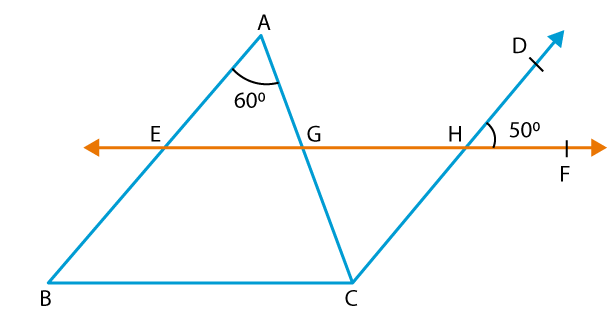Solution:

We know that AB || CD and AC is a transversal

From the figure we know that ∠BAC and ∠ACD are alternate angles

So we get

∠BAC = ∠ACD = 60o

So we also get

∠BAC = ∠GCH = 60o

From the figure we also know that ∠DHF and ∠CHG are vertically opposite angles

So we get

∠DHF = ∠CHG = 50o

We know that the sum of all the angles in triangle GCH is 180o.

So we can write it as

∠GCH + ∠CHG + ∠CGH = 180o

By substituting the values

60o + 50o + ∠CGH = 180o

On further calculation

∠CGH = 180o – 60o – 50o

By subtraction

∠CGH = 180o – 110o

∠CGH = 70o

From the figure we know that ∠CGH and ∠AGH form a linear pair of angles

So we get

∠CGH + ∠AGH = 180o

By substituting the values

70o + ∠AGH = 180o

On further calculation

∠AGH = 180o – 70o

By subtraction

∠AGH = 110o

Therefore, ∠GCH = 60o and ∠AGH = 110o.

18. Calculate the value of x in the given figure.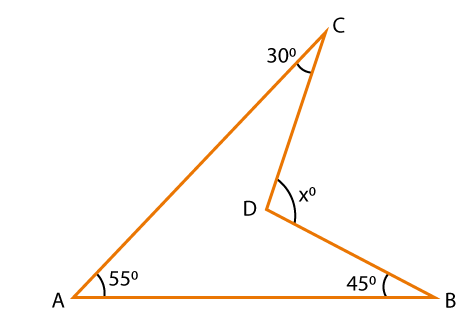Solution:

Construct a line CD to cut the line AB at point E.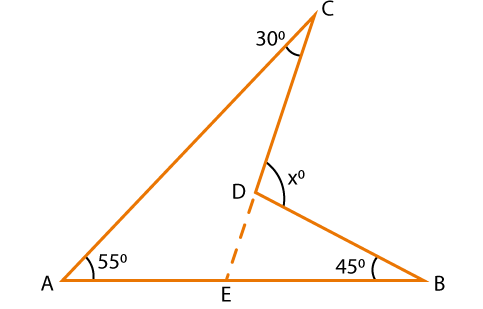We know that in the triangle BDE exterior angle is equal to the sum of two opposite interior angles

So we can write it as

∠CDB = ∠CEB + ∠DBE

By substituting the values

xo = ∠CEB + 45o …. (1)

We know that in the triangle AEC exterior angle is equal to the sum of two opposite interior angles

So we can write it as

∠CEB = ∠CAB + ∠ACE

By substituting the values

∠CEB = 55o + 30o

∠CEB = 85o

By substituting ∠CEB in equation (1) we get

xo = 85o + 45o

xo = 130o

19. In the given figure, AD divides ∠BAC in the ratio 1: 3 and AD = DB. Determine the value of x.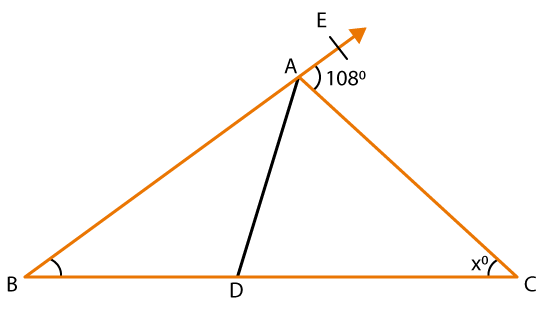Solution:

It is given that AD divides ∠BAC in the ratio 1: 3

So let us consider ∠BAD and ∠DAC as y and 3y

According to the figure we know that BAE is a straight line

From the figure we know that ∠BAC and ∠CAE form a linear pair of angles

So we get

∠BAC + ∠CAE = 180o

We know that

So it can be written as

∠BAD + ∠DAC + ∠CAE = 180o

By substituting the values we get

y + 3y + 108o = 180o

On further calculation

4y = 180o – 108o

By subtraction

4y = 72o

By division

y = 72/4

y = 18o

We know that the sum of all the angles in triangle ABC is 180o.

So we can write it as

∠ABC + ∠BCA + ∠BAC = 180o

It is given that AD = DB so we can write it as ∠ABC = ∠BAD

From the figure we know that ∠BAC = y + 3y = 4y

By substituting the values

y + x + 4y = 180o

On further calculation

5y + x = 180o

By substituting the value of y

5 (18o) + x = 180o

By multiplication

90o + x = 180o

x = 180o – 90o

By subtraction we get

x = 90o

Therefore, the value of x is 90.

20. If the sides of a triangle are produced in order, prove that the sum of the exterior angles so formed is equal to four right angles.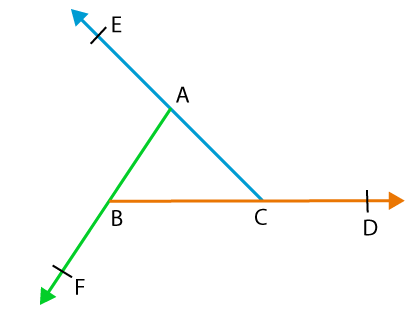Solution:

Given: △ ABC in which AB, BC and CA are produced to points D, E and F.

To prove: ∠DCA + ∠FAE + ∠FBD = 180o

Proof:

From the figure we know that

∠DCA = ∠A + ∠B …. (1)

∠FAE = ∠B + ∠C …. (2)

∠FBD = ∠A + ∠C …. (3)

By adding equation (1), (2) and (3) we get

∠DCA + ∠FAE + ∠FBD = ∠A + ∠B + ∠B + ∠C + ∠A + ∠C

So we get

∠DCA + ∠FAE + ∠FBD = 2 ∠A + 2 ∠B + 2 ∠C

Now by taking out 2 as common

∠DCA + ∠FAE + ∠FBD = 2 (∠A + ∠B + ∠C)

We know that the sum of all the angles in a triangle is 180o.

So we get

∠DCA + ∠FAE + ∠FBD = 2 (180o)

∠DCA + ∠FAE + ∠FBD = 360o

Therefore, it is proved.

21. In the adjoining figure, show that ∠A + ∠B +∠C + ∠D + ∠E + ∠F = 360o.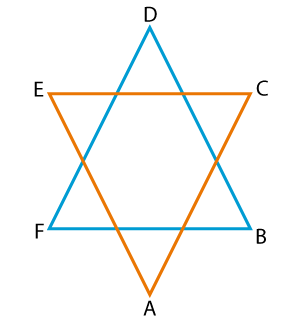Solution:

We know that the sum of all the angles in triangle ACE is 180o.

∠A + ∠C + ∠E = 180o ….. (1)

We know that the sum of all the angles in triangle BDF is 180o.

∠B + ∠D + ∠F = 180o ….. (2)

Now by adding both equations (1) and (2) we get

∠A + ∠C + ∠E + ∠B + ∠D + ∠F = 180o + 180o

On further calculation

∠A + ∠B + ∠C + ∠D + ∠E + ∠F = 360o

Therefore, it is proved that ∠A + ∠B + ∠C + ∠D + ∠E + ∠F = 360o.

22. In the given figure, AM ⊥ BC and AN is the bisector of ∠A. If ∠ABC = 70o and ∠ACB = 20o, find ∠MAN.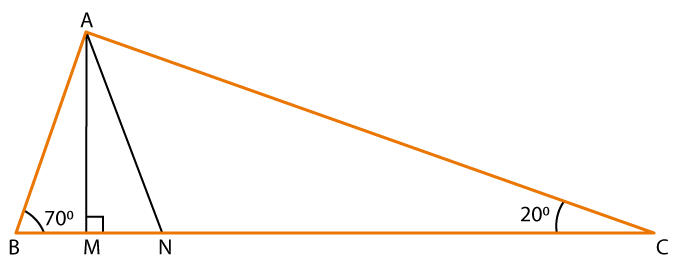Solution:

We know that the sum of all the angles in triangle ABC is 180o.

∠A + ∠B + ∠C = 180o

By substituting the values

∠A + 70o + 20o = 180o

On further calculation

∠A = 180o – 70o – 20o

By subtraction

∠A = 180o – 90o

∠A = 90o

We know that the sum of all the angles in triangle ABM is 180o.

∠BAM + ∠ABM + ∠AMB = 180o

By substituting the values

∠BAM + 70o + 90o = 180o

On further calculation

∠BAM = 180o – 70o – 90o

By subtraction

∠BAM = 180o – 160o

∠BAM = 20o

It is given that AN is the bisector of ∠A

So it can be written as

∠BAN = (1/2) ∠A

By substituting the values

∠BAN = (1/2) (90o)

By division

∠BAN = 45o

From the figure we know that

∠MAN + ∠BAM = ∠BAN

By substituting the values we get

∠MAN + 20o = 45o

On further calculation

∠MAN = 45o – 20o

By subtraction

∠MAN = 25o

Therefore, ∠MAN = 25o.

23. In the given figure, BAD || EF, ∠AEF = 55o and ∠ACB = 25o, find ∠ABC.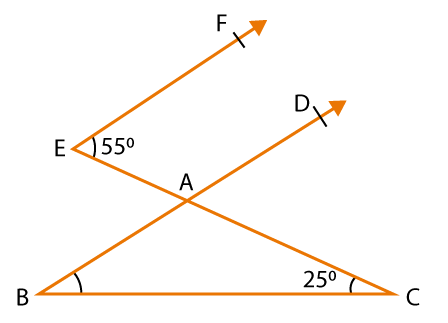Solution:

We know that BAD || EF and EC is the transversal

From the figure we know that ∠AEF and ∠CAD are corresponding angles

So we get

From the figure we know that ∠CAD and ∠CAB form a linear pair of angles

So we get

By substituting the values

55o + ∠CAB = 180o

On further calculation

∠CAB = 180o – 55o

By subtraction

∠CAB = 125o

We know that the sum of all the angles in triangle ABC is 180o.

∠ABC + ∠CAB + ∠ACB = 180o

By substituting the values in the above equation we get

∠ABC + 125o + 25o = 180o

On further calculation

∠ABC = 180o – 125o – 25o

By subtraction

∠ABC = 180o – 150o

∠ABC = 30o

24. In a △ ABC, it is given that ∠A: ∠B: ∠C = 3: 2: 1 and CD ⊥ AC. Find ∠ECD.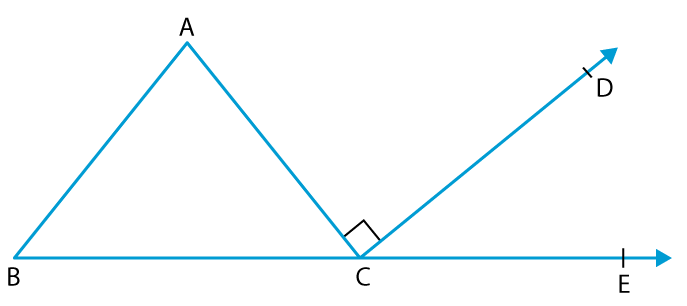Solution:

In a △ ABC, it is given that

∠A: ∠B: ∠C = 3: 2: 1

It can also be written as

∠A = 3x, ∠B = 2x and ∠C = x

We know that the sum of all the angles in triangle ABC is 180o.

∠A + ∠B + ∠C = 180o

By substituting the values we get

3x + 2x + x = 180o

6x = 180o

By division

x = 180/6

x = 30o

Now by substituting the value of x we get

∠A = 3x = 3 (30o) = 90o

∠B = 2x = 2 (30o) = 60o

∠C = x = 30o

We know that in the triangle ABC exterior angle is equal to the sum of two opposite interior angles

So we can write it as

∠ACE = ∠A + ∠B

By substituting the values we get

∠ACE = 90o + 60o

∠ACE = 150o

We know that ∠ACE can be written as ∠ACD + ∠ECD

So we can write it as

∠ACE = ∠ACD + ∠ECD

By substituting the values we get

150o = 90o + ∠ECD

It is given that CD ⊥ AC so ∠ACD = 90o

On further calculation

∠ECD = 150o – 90o

By subtraction

∠ECD = 60o

Therefore, ∠ECD = 60o.

25. In the given figure, AB || DE and BD || FG such that ∠ABC = 50o and ∠FGH = 120o. Find the values of x and y.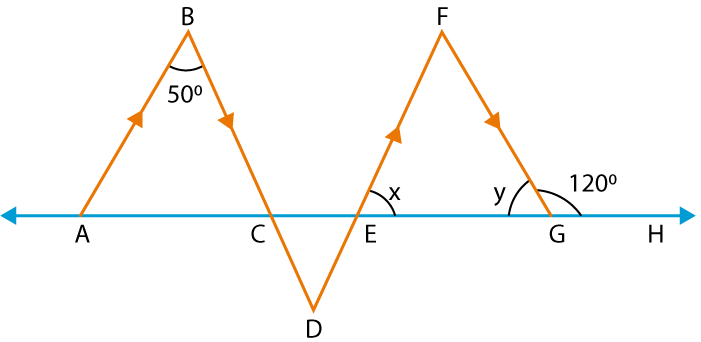Solution:

From the figure we know that ∠FGH and ∠FGE form a linear pair of angles

So we get

∠FGH + ∠FGE = 180o

By substituting the values

120o + y = 180o

On further calculation

y = 180o – 120o

By subtraction

y = 60o

We know that AB || DF and BD is a transversal

From the figure we know that ∠ABC and ∠CDE are alternate angles

So we get

∠ABC = ∠CDE = 50o

We know that BD || FG and DF is the transversal

From the figure we know that ∠EFG and ∠CDE are alternate angles

So we get

∠EFG = ∠CDE = 50o

We know that the sum of all the angles in triangle EFG is 180o.

∠FEG + ∠FGE + ∠EFG = 180o

By substituting the values we get

x + y + 50o = 180o

x + 60o + 50o = 180o

On further calculation

x = 180o – 60o – 50o

By subtraction

x = 180o – 110o

x = 70o

Therefore, the values of x = 70o and y = 60o.

26. In the given figure, AB || CD and EF is a transversal. If ∠AEF = 65o, ∠DFG = 30o, ∠EGF = 90o and ∠GEF = xo, find the value of x.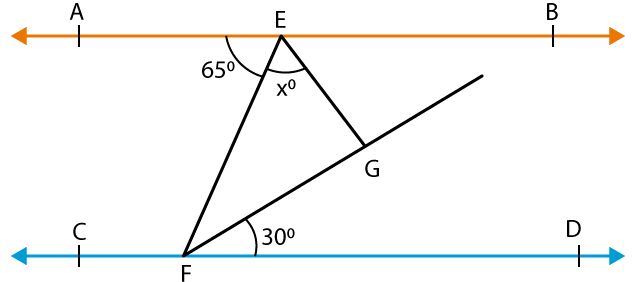Solution:

We know that AB || CD and EF is a transversal

From the figure we know that ∠AEF and ∠EFD are alternate angles

So we get

∠AEF = ∠EFG + ∠DFG

By substituting the values

65o = ∠EFG + 30o

On further calculation

∠EFG = 65o – 30o

By subtraction

∠EFG = 35o

We know that the sum of all the angles in triangle GEF is 180o.

∠GEF + ∠EGF + ∠EFG = 180o

By substituting the values we get

x + 90o + 35o = 180o

On further calculation

x = 180o – 90o – 35o

By subtraction

x = 55o

Therefore, the value of x is 55o

27. In the given figure, AB || CD, ∠BAE = 65o and ∠OEC = 20o. Find ∠ECO.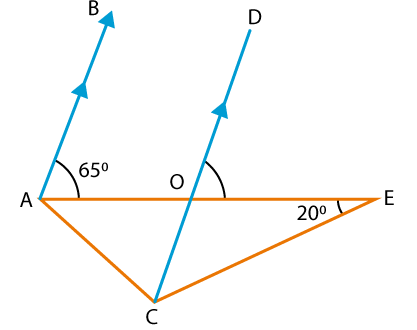Solution:

We know that AB || CD and AE is a transversal

From the figure we know that ∠BAE and ∠DOE are corresponding angles

So we get

∠BAE = ∠DOE = 65o

From the figure we know that ∠DOE and ∠COE form a linear pair of angles

So we get

∠DOE + ∠COE = 180o

By substituting the values

65o + ∠COE = 180o

On further calculation

∠COE = 180o – 65o

By subtraction

∠COE = 115o

We know that the sum of all the angles in triangle OCE is 180o.

∠OEC + ∠ECO + ∠COE = 180o

By substituting the values we get

20o + ∠ECO + 115o = 180o

On further calculation

∠ECO = 180o – 20o – 115o

By subtraction

∠ECO = 45o

Therefore, ∠ECO = 45o

28. In the given figure, AB || CD and EF is a transversal, cutting them at G and H respectively. If ∠EGB = 35o and QP ⊥ EF, find the measure of ∠PQH.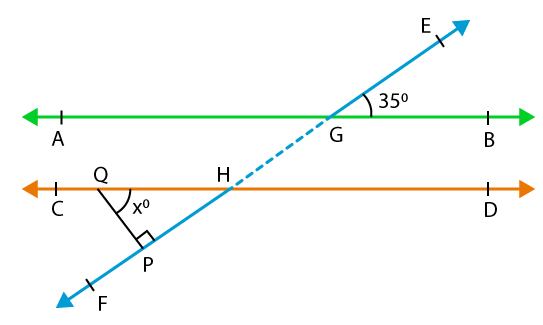Solution:

We know that AB || CD and EF is a transversal

From the figure we know that ∠EGB and ∠GHD are corresponding angles

So we get

∠EGB = ∠GHD = 35o

From the figure we know that ∠GHD and ∠QHP are vertically opposite angles

So we get

∠GHD = ∠QHP = 35o

We know that the sum of all the angles in triangle DQHP is 180o.

∠PQH + ∠QHP + ∠QPH = 180o

By substituting the values we get

∠PQH + 35o + 90o = 180o

On further calculation

∠PQH = 180o – 35o – 90o

By subtraction

∠PQH = 180o – 125o

∠PQH = 55o

Therefore, ∠PQH = 55o

29. In the given figure, AB || CD and EF ⊥ AB. If EG is the transversal such that ∠GED = 130o, find ∠EGF.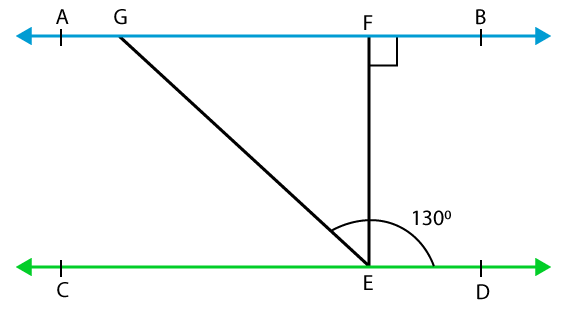Solution:

We know that AB || CD and GE is the transversal

From the figure we know that ∠EGF and ∠GED are interior angles

So we get

∠EGF + ∠GED = 180o

By substituting the values

∠EGF + 130o = 180o

On further calculation

∠EGF = 180o – 130o

By subtraction

∠EGF = 50o

Therefore, ∠EGF = 50o

### RS Aggarwal Solutions for Class 9 Maths Chapter 8: Triangles

Chapter 8, Triangles, has 1 exercise with solutions prepared by our expert team of faculties having much knowledge about the concepts in Mathematics. Some of the topics in RS Aggarwal Solutions which are explained in brief under this chapter are:

• Triangles
• Types of triangles on the basis of sides
• Types of triangles on the basis of angles
• Some terms related to triangle
• Exterior and interior opposite angles of a triangle
• Some results on triangles

### RS Aggarwal Solutions Class 9 Maths Chapter 8 – Triangles

Students can make use of the RS Aggarwal Solutions which covers the entire syllabus as per the textbook with exercise wise answers in each chapter. The brief solutions prepared by our experts mainly help students to solve the problems of higher difficulty with ease. RS Aggarwal Solutions for Class 9 can be used by the students as a vital resource to boost their exam preparation. Some of the applications of triangles are architecture, design of buildings, height of bridges and finding the area of geometric structures. Here, we provide PDF containing chapter wise solutions of the entire chapter based on the RS Aggarwal textbook to help students study well before appearing for the exams.